一些关于技术的小小思考。

# 前言

实习返校已经有一个月啦，但是返校后实在是太忙了。最近也在调整状态中，顺手写一下技术的复盘（复盘 拉通 对齐 ~ 互联网黑话学废了（狗头保命））。

能够得到算法实习机会，还是我读研后一直在研究的推荐算法岗，我非常幸运。

回忆三个月的实习生活，短暂又充实。还记得拿到实习offer时发现竟是在全上海最繁华的地段工作，感觉捡到宝了哈哈哈哈。

感受了一把互联网企业的作息时间10-8-5（感谢mentor没有让我周六加班），了解了真正的算法工程师每天是怎么干活的，和开发岗还是有很多不一样的地方。

实习前幻想中的算法工程师的日常：
天天看顶会paper，复现模型，科研工程两手抓，Python代码玄学魔改，工程师个个头发茂密穿着最常见的格子衬衣带着眼镜研讨模型应该再加个什么类型的注意力机制（雾），IT精英的代表，算法人上人（滑稽）！

现实中的算法工程师的日常：

数据——不想当优秀数据清洁工的算法工程师不是合格的程序员。

模型——先上简单的，能用再说。后期可以慢慢调。

指标——人工评估yyds。

以上多少带点开玩笑的语气，大佬们看了不要拍我呀（嘻嘻）

下面是正经地技术思考贴！

# 算法 vs 开发

算法分为很多种的，比如通信算法、机械算法、人工智能向算法，我这里主要说的是人工智能向的算法。人工智能向的算法，粗略地说往往称为机器学习算法，里面又包括深度学习算法。按不同方向有两大类需求最多，一类是视觉CV算法，一类是NLP算法，这是根据要实现的任务进行分类。还有根据场景进行分类的，比如推荐算法、广告算法、搜索算法。我谈谈推荐算法岗。

搜广推在很多方面是有共通之处的，所以我们常常说搜广推是一家，当然，具体还是有业务差异的。推荐算法在几年是得到了飞速发展的，尤其是深度学习时代来临以后，各种在DNN上的魔改开始广泛出现在业务上，而不再是LR一把梭。

可能每个在学校做科研的学生都把模型视作解决问题的关键，毕竟顶会里介绍模型的彩色图片制作精美，结构复杂，高端大气上档次，让人看了都要感叹一声这就是前沿技术的魅力吗？我在实习前，也天天想着怎么魔改我论文里的模型，让它看上去再高级一点，跟得上AI时代一些，即使我导已经点出了模型的创新是比较难的，而我依然执着于此，妄想创造个如同bert一样惊世骇俗的模型出来。

实习中给我最大的收获是，算法工程师最核心的能力除了对各种模型的熟练以外，在数据侧的研究也要捻熟于心。

数据！数据！数据！

事实就是，想破脑袋去魔改模型或者玄学调参，很有可能不如认真检查数据问题对结果提升来得快。我现在可以理解，为什么很多人把算法工程师划分到数据科学里了。现在的机器学习工程师，狭隘地说，就是借工具来挖掘数据的规律，进而实现一定的预测能力。

开发则更偏向于具体实现。如果说开发是一个人的躯干，那么算法则是一个人的灵魂。灵魂不能脱离躯干，所以算法离不开开发。但是很多小公司不需要算法，正如只需要工具人干活就好了，工具人不需要灵魂。大厂想做有理想的产品，能改变世界的产品，就会需要灵魂去创新，也就需要算法。

开发和算法的成就感也截然不同。开发的成就感更多来自于模块或者系统编写成功，能够运行带来的快感。想着成千上万的用户正在使用我写的软件，这种存在感是成就感的来源。算法的成就感往往来自指标的提升，比如广告部门赚钱更多了，点击率更多了，转化率更多了，从而推测我们的算法起作用了。开发很多时候是在重复造轮子，而算法有时候是在寻找一个没有经验可循的新方法，这时脑洞和经验齐飞，创新不再是空谈。

这几年来，我对算法和开发都有了若干的尝试，这对于我曾经向往的全栈有了很好的打底，想必未来在分析一个问题的时候，从开发和算法不同的角度想方案，会有不一样的思索。

# 编程语言之争

一直以来，关于到底学什么编程语言好的争论从未停止过。我自己从接触开发开始，也经历过很多次摇摆。本科时最早学习的Java，毕设做的安卓app，结果第一份工作阴差阳错做的C++软件开发。后来读研，又开始做算法科研，用的Python。为了接触大数据，又重新学习Java。

曾经我也不断问自己，到底选C++还是Java？C++偏底层，更适合于服务器开发、游戏开发、算法引擎等，Java更适合于Web、企业级应用等。我到底喜欢哪个？

直到在实习过程中，我发现即使我是算法工程师，写Python为主，可是要上线的时候，我还是要写Java的。

突然就真正地意识到，编程语言只是工具，面对什么场景，什么语言合适就用什么语言，而不是我喜欢什么语言写什么语言。我为什么不能既会Java又会C++还能写Python呢？

其实只要学会一种编译语言，再学会一种脚本语言，基本上要迁移到其它语言是不难的。甚至现在刷题的时候，看到一个问题，可能也不一定限制于用某一种语言写，而是会考虑哪一种语言最适合这道题，就用哪种语言做。

啊，我悟了。

# 总结

最近一两年纠结的两大问题：选算法还是选开发，选C++还是选Java，在上面复盘了一遍，说了又好像没有完全说，哈哈哈哈哈。

总之，写代码的活就是最好的！

闭环了。

]]>
<div align="left"><br><img src="\images\假装有图片.jpg" width="300" height="180" style="float:right;"><br><br><br>　　<strong>一些关于技术的小小思考。</strong><br><br><br> </div>效率在大数据量下是非常重要的。

Iteratively appending rows to a DataFrame can be more computationally intensive than a single concatenate. A better solution is to append those rows to a list and then concatenate the list with the original DataFrame all at once.

]]>
<div align="left"><br><img src="\images\cover-pandas.jpg" width="300" height="180" style="float:right;"><br><br><br><br>　　<strong>效率在大数据量下是非常重要的。</strong><br><br><br> </div>
Pandas自用笔记 https://hubojing.github.io/2021/07/11/Pandas杂记/ 2021-07-11T08:02:53.000Z 2021-07-11T15:23:31.580Z代码不写就会忘
笔记不整理就会乱

# 参考文献

pandas官方文档

python中groupby函数详解（非常容易懂）

[Python3]pandas.merge用法详解

]]>
<div align="left"><br><img src="\images\cover-pandas.jpg" width="300" height="180" style="float:right;"><br><br><br><br>　　<strong>代码不写就会忘</strong><br>　　<strong>笔记不整理就会乱</strong><br><br><br> </div>
CRF++ https://hubojing.github.io/2021/06/16/CRF++/ 2021-06-16T14:31:37.000Z 2021-06-16T15:13:02.950Z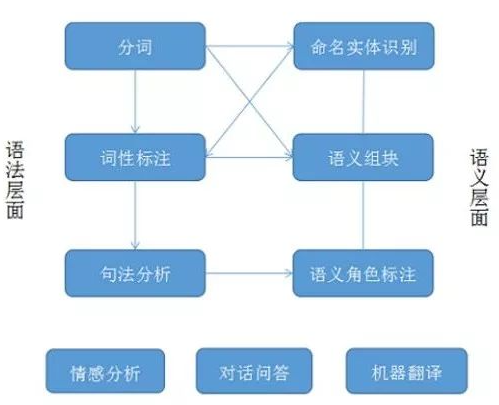CRF++笔记。

# NLP引入

句法分析是NLP任务的核心，NER是句法分析的基础。NER任务用于识别文本中的人名（PER）、地名（LOC）等具有特定意义的实体。非实体用O来表示。

# CRF

Conditional Random Field，条件随机场，一种机器学习模型，广泛用于NLP文本标注领域。
应用场景：分词、词性标注、命名实体识别（NER，Named Entity Recognition）等。
命名实体识别的任务是识别出待处理文本中三大类（实体类、时间类和数字类）、七小类（人名、机构名、地名、时间、日期、货币和百分比）命名实体。

NER是个分类任务,具体称为序列标注任务，即文本中不同的实体对应不同的标签，人名-PER，地名-LOC，等等，相似的序列标注任务还有词性标注、语义角色标注。

传统解决方法：
（1）基于规则
（2）基于统计学
隐马尔可夫（HMM）、条件随机场（CRF）模型和Viterbi算法
（3）神经网络
LSTM+CRF模型

CRF的基础是马尔科夫随机场（概率无向图）。
CRF可以理解为在给定随机变量X的条件下，随机变量Y的马尔可夫随机场。其中，线性链CRF（一种特殊的CRF）可以用于序列标注问题。CRF模型在训练时，给定训练序列样本集(X,Y)，通过极大似然估计、梯度下降等方法确定CRF模型的参数；预测时，给定输入序列X，根据模型，求出P(Y|X)最大的序列y。

## CRF分词原理

CRF把分词当做字的词位分类问题，通常定义字的词位信息如下：
词首，常用B表示
词中，常用M表示
词尾，常用E表示
单子词，常用S表示

例：
原始例句：他爱上海陆家嘴
CRF标注后：他/S 爱/S 上/B 海/E 陆/B 家/M 嘴/E
分词结果：他/爱/上海/陆家嘴

用CRF进行命名实体识别属于有监督学习。需要大批已标注的语料对模型参数进行训练。

# CRF++

目前常见的CRF工具包有pocket crf, flexcrf 和CRF++。
CRF++是著名的条件随机场的开源工具，也是目前综合性能最佳的CRF工具。
CRF++官网http://taku910.github.io/crfpp/

## 安装

1. 解压
2. cd 进入解压后的目录，执行‘./configure’命令
3. make 编译
4. make install （需先执行“su”获取root用户权限）
5. make clean 删除安装时产生的临时文件（可不执行）

## 使用

两个过程：训练、测试。

### 数据格式

训练和测试文件必须包含多个tokens，每个token又包含多个列。token的定义可根据具体的任务，如词、词性等。每个token必须写在一行，且各列之间用空格或制表格间隔。一个token的序列可构成一个sentence，每个sentence之间用一个空行间隔。注意最后一列将是被CRF用来训练的最终标签。

### 特征模板

CRF++训练的时候，要求我们自己提供特征模板。
模板文件中的每一行是一个模板。每个模板都是由%x[row,col]来指定输入数据中的一个token。row指定到当前token的行偏移，col指定列位置。

# 参考文献

]]>
<div align="left"><br><img src="\images\CRF++_NLP流程.png" width="300" height="180" style="float:right;"><br><br><br>　　<strong>CRF++笔记。</strong><br><br><br> </div>
【Paper】DIN模型 https://hubojing.github.io/2021/05/06/DIN/ 2021-05-06T02:55:35.000Z 2021-10-21T09:03:21.000Z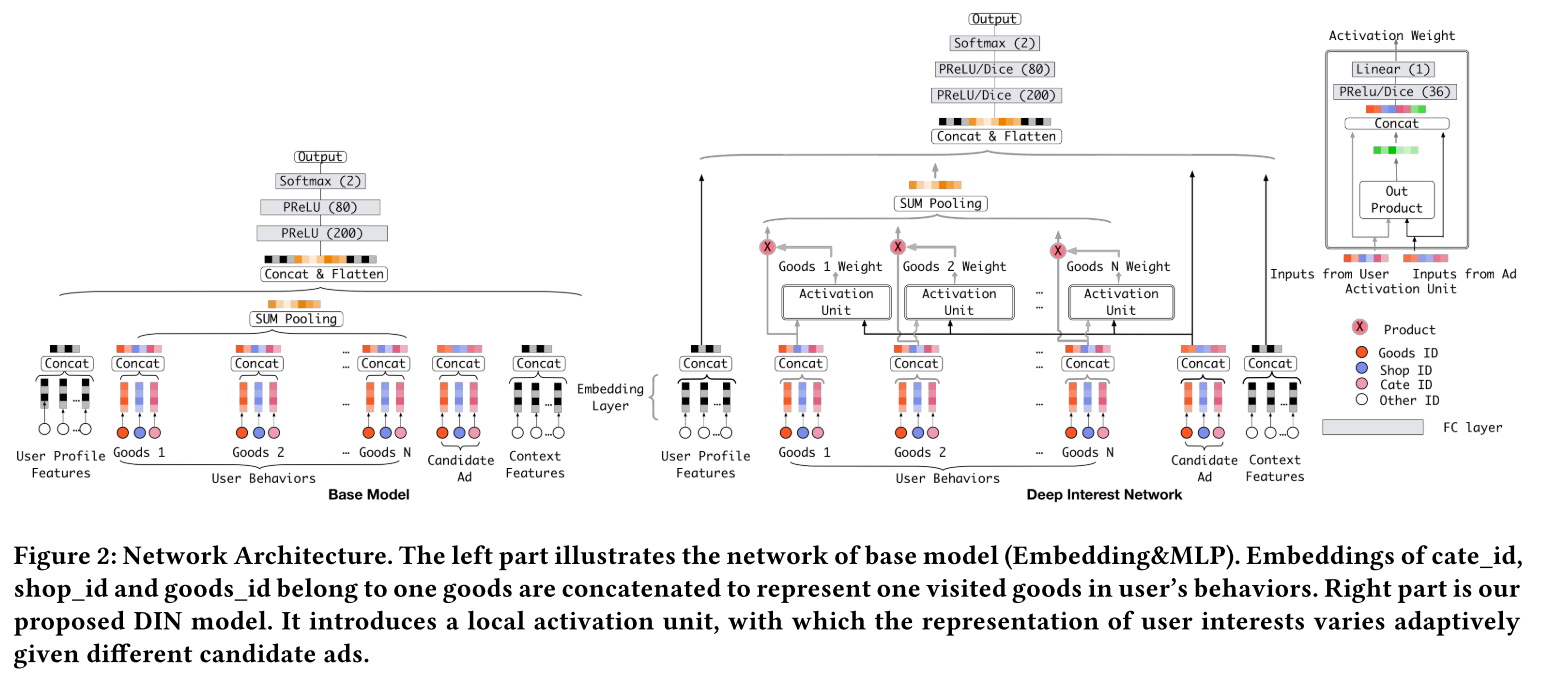阿里DIN模型
10.21总算写完了……

2018年 阿里

# 现有问题

目前的深度学习模型都是先将稀疏输入特征映射为低维嵌入向量，再转换为固定长度的向量，最后联结起来送入MLP。这个固定长度的向量会成为瓶颈，无法从历史行为中捕获用户不同的兴趣。因此，本文提出深度兴趣网络Deep Interest Network（DIN）。它设计了一个局部激活单元从用户历史行为中自适应学习用户兴趣。另外，本文提出了两大技术：小批量感知正则化（mini-batch aware regularization）和数据自适应激活函数（data adaptive activation function）。

# 引言 INTRODUCTION

Embedding & MLP方法通过将用户行为嵌入向量转换为一个固定长度的向量来学习用户所有兴趣的表示，所有的表示向量是欧式空间。换言之，将用户不同的兴趣压缩到一个固定长度的向量，限制了表达能力。为了更好地表达用户不同兴趣，就要扩展向量长度。这会增多学习参数，并且增加过拟合的风险。也加重了计算和存储的压力，对于工业线上系统来说很困难。
另一方面，没有必要把用户全部兴趣压缩到同一个向量里，因为只有部分兴趣会影响用户下一个动作（点击或不点击）。

训练的问题：
基于SGD的优化方法只更新出现在每个小批量中的稀疏特征的参数。然而，加上传统的ℓ2正则化，计算变得不可接受，这需要为每个小批量计算整个参数的L2范数(在阿里的场景，大小按比例增加到数十亿)。本文提出了一种新的小批量正则化方法，在L2范数的计算中，每个小批量正则化中只出现非零特征参数，使得计算是可接受的。另外，设计了一个数据自适应激活函数，推广到常用的PReLU，它通过自适应地调整输入的校正点，也就是输入的分布，并被证明有助于训练具有稀疏特征的工业网络。

1. 指出了使用固定长度向量来表达用户不同兴趣的局限性，并设计了一种新的深度兴趣网络(DIN)，它引入了一个局部激活单元来自适应地从给定广告的历史行为中学习用户兴趣的表示。DIN可以大大提高模型的表达能力，更好地捕捉用户兴趣的多样性特征。
2. 开发了两种新的技术来帮助训练工业深度网络:I)一种小批量感知正则化器，这种正则化器在具有大量参数的深度网络上节省了大量的正则化计算，并且有助于避免过拟合；ii)一种数据自适应激活函数，这种函数通过考虑输入的分布来推广PReLU，并且表现出良好的性能。
3. 在公共数据集和AI-ibaba数据集上进行了大量实验。结果验证了所提出的DIN和训练技术的有效性。所提出的方法已经在全球最大的广告平台之一阿里巴巴的商业展示广告系统中得到了应用，为业务的发展做出了重大贡献。

# 深度兴趣网络 DEEP INTEREST NETWORK

## 特征表示 Feature Representation

表中描述了我们系统中使用的全部特征集，它由四类组成，其中用户行为特征是典型的多热点编码向量，包含丰富的用户兴趣信息。注意，在我们的设置中，没有组合特性。我们利用深度神经网络捕获特征的交互作用。

## 基线模型 Base Model(Embedding&MLP)

得到定长向量：$e_i = pooling(e_{i_1}, e_{i_2}, …, e_{i_k})$

最常用的是sum pooling和average pooling。

MLP

Loss

$$L = - \frac{1}{N}\sum_{(x,y)∈S}(ylogp(x) + (1 - y)log(1 - p(x)))$$

S是尺寸为N的训练集，x是输入，y是标签。p(x)是经过softmax层后的输出，代表样本x被点击的概率。

## DIN架构 The structure of Deep Interest Network

基线模型对一个用户使用定长向量表示，无论候选广告是什么。

为了表示用户兴趣多样化，一种简单的方法是扩展嵌入维度，但这会增加学习参数规模。有过拟合的风险，并且增加了计算和存储的负担。

DIN模拟了关于给定广告的用户局部激活兴趣。

DIN引入了一个用于用户行为特征的局部激活单元，使用了加权求和池化（weighted sum pooling）来自适应计算当给定一个候选广告A时，用户的表示$V_U$。

局部激活单元公式：
$$v_U = f(v_A, e_1, e_2, …, e_H) = \sum_{j=1}^{H}a(e_j, v_A)e_j = \sum_{j = 1}^{H}w_je_j$$
其中，${e_1, e_2, …, e_H}$是用户U的行为嵌入向量（长度为H），$v_U(A)$是广告A的嵌入向量。
在这种方式下，$v_U(A)$在不同的广告下，a(·)是一个带有激活权重输入的前向反馈网络。除了这两部分输入嵌入向量，a(·)将它们的外积喂入后续网络。
a(·)softmax输出后的标准化被舍弃。

# 训练方法 TRAINING TECHNIQUES

## 小批量感知正则化 Mini-batch Aware Regularization

工业训练网络面临过拟合的问题。模型训练在第一轮训练后（不加正则化）性能迅速下降。传统的正则策略在面对稀疏输入和成千上万的参数时并不适用（比如l2和l1正则化)。
为此，提出小批量感知正则化，即只在每次小批量中对稀疏特征的参数计算$L_2-norm$。

$$L_2(W)=||W||_{2}^{2}$$

$$= \sum_{j = 1}^{K}||w_j||_{2}^{2}$$

$$= \sum_{(x,y)∈S}\sum_{j = 1}^{K}\frac{I(x_j≠0)}{n_j}||w_j||_2^2$$

$I(x_j≠0)$表示x有特征j，$n_j$表示特征id j出现的数量。上述公式可以被转换为下面的小批量感知形式：

$$L_2(W) = \sum_{j = 1}^{K}\sum_{m = 1}^{B}\sum_{(x,y)∈B_m}\frac{I(x_j≠0)}{n_j}||w_j||_2^2$$

其中，B表示小批量的数量，$B_m$表示第m次小批量。定义$α{mj} = max{(x,y)∈B_m}I(x_j ≠ 0)$为小批量$B_m$中至少有一次有特征id j。
上述近似为

$$L_2(W) ≈ \sum_{j = 1}^{K}\sum_{m = 1}^{B}\frac{α_{mj}}{n_j}||w_j||_2^2$$

对于第m次小批量，关于特征j的嵌入权重的梯度为

$$w_j\leftarrow w_j - \eta[\frac{1}{|B_m|}\sum_{(x,y)∈B_m}\frac{\partial L(p(x), y)}{\partial w_j} + \lambda \frac{α_{mj}}{n_j}w_j]$$

在这里只有出现在第m次小批量的特征参数才会参与正则计算。

## 数据自适应激活函数 Data Adaptive Activation Function

PReLU是常用的激活函数。

如图所示，PReLU在0附近有一个固定的转折点，这对于每层不同分布的输入是不合适的。于是，本文设计了一个自适应激活函数Dice。
$$f(s) = p(s)·s + (1-p(s))·αs, p(s) = \frac{1}{1 + e^{-\frac{s-E[s]}{\sqrt{Var[s] + \epsilon}}}}$$
E[s]和Var[s]代表每次小批量输入的均值和方差。$\epsilon$是一个常量，此处设为$10^{-8}$。
Dice的主要思想是根据输入数据分布自适应调整转折点，值设置为输入的平均值。另外，Dice在两个函数间切换很顺滑。当E(s) = 0且Var[s] = 0时，Dice退化到PReLU。

# 实验 EXPERIMENTS

## 数据集和实验步骤 Datasets and Experimental Setup

数据集三个：Amazon Dataset， MovieLens Dataset和Alibaba Dataset。
Amazon Dataset：http://jmcauley.ucsd.edu/data/amazon/
MovieLens Dataset：https://grouplens.org/datasets/movielens/20m/
数据集情况如图。

• LR
• BaseModel
• Wide&Deep
• PNN
• DeepFM

## 策略

$$AUC = \frac{\sum_{i = 1}^{n}impression_i \times AUC_i}{\sum_{i = 1}^{n}impression_i}$$

$$RelaImpr = (\frac{AUC(measured model) - 0.5}{AUC(base model) - 0.5} - 1) \times 100\%$$
RelaImpr用来衡量模型间的相对提升。

如图所示。

## 正则化性能 Performance of regularization

对比试验：

• Dropout
• Filter
• Regularization in DiFacto
• MBA

# 总结 CONCLUSIONS

传统CTR模型适用定长向量代表用户兴趣是有缺陷的。为了提高用户兴趣多样性，提出DIN模型来激活相关的用户行为，并且针对不同的广告有一个自适应用户兴趣表示向量。另外，提出两种技术帮助训练工业深度网络，并提升了DIN性能。DIN已被部署到阿里巴巴的在线广告展示系统。
]]>
<div align="left"><br><img src="\images\DIN架构.png" width="300" height="180" style="float:right;"><br><br><br>　　<strong>阿里DIN模型</strong><br>　　<strong>10.21总算写完了……</strong><br><br><br> </div>
【Paper】Entire Space Multi-Task Model-An Effective Approach for Estimating Post-Click Conversion Rate https://hubojing.github.io/2021/04/27/ESMM/ 2021-04-27T02:23:10.000Z 2021-05-06T09:39:02.875Z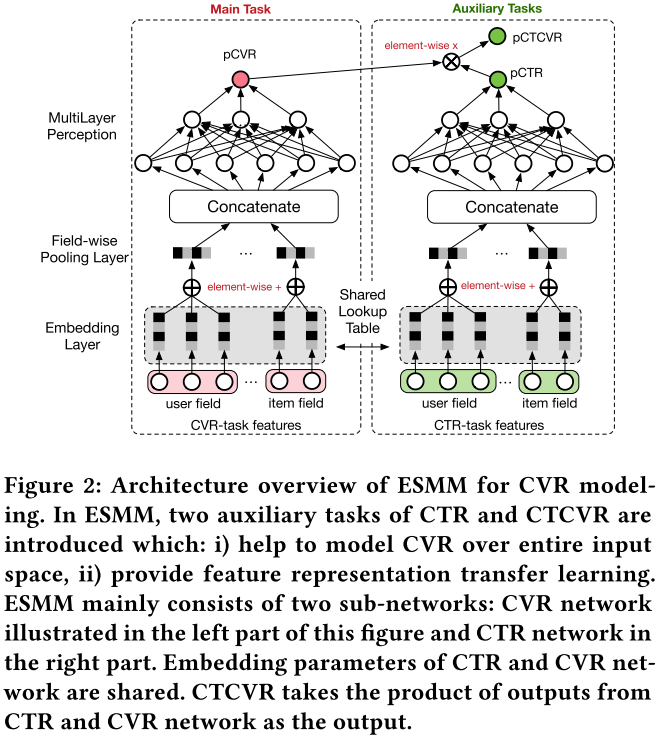多任务学习之ESMM

2018年 阿里巴巴

# 介绍 INTRODUCTION

1）传统的CVR模型在由impression组成的数据集上训练，同时利用所有impression的样本在整个空间上进行推断。该问题（SSB）会影响训练模型的泛化性能。
2）数据稀疏问题。在实践中，为训练CVR模型而收集的数据通常比CTR任务少得多。训练数据的稀疏性使得CVR模型拟合相当困难。

pCVR = p(conversion|click, impression)

ESMM可以同时解决SSB和DS问题。它引入了CTR和CTCVR的两个辅助任务。ESMM没有直接用曝光的样本来训练CVR模型，而是把pCVR作为一个中间变量，乘以pCTR等于pCTCVR。PCTCVR和pCTR都是在整个空间上用所有曝光的样本来估计的，因此导出的pCVR也适用于整个空间。这表明SSB问题已经消除。此外，CVR网络特征表示的参数与CTR网络共享。后者是用更丰富的样本训练的。这种参数迁移学习有助于显著缓解DS问题。

# 提出的方法 THE PROPOSED APPROACH

## 注释 Notation

$S = {(x_i, y_i -> z_i)}|^N_{i=1}$，x代表已观察曝光的特征向量，它通常是一个有多域(field)的高维稀疏向量，比如用户域，物品域等。y和z是二分标签，y = 1或者z = 1代表各自被点击或转化事件发生。y->z代表点击和转化标签的序列依赖，即转化事件发生时总会有前序点击。
pCVR = p(z = 1|y = 1, x)
pCTR = p(y = 1|x)
pCTCVR = p(y = 1, z = 1|x)
p(y = 1, z = 1|x) = p(y = 1|x) × p(z = 1|y = 1, x)
—–pCTCVR————pCTR———–pCVR——–

## CVR建模和挑战 CVR Modeling and Challenges

Sample selection bias(SSB)

Data sparsity (DS)

## 多任务全空间模型 Entire Space Multi-Task Model

$p(z = 1|y = 1, x) = \frac{p(y = 1, z = 1|x)}{p(y = 1|x)}$

l(·)是交叉熵损失函数。

]]>
<div align="left"><br><img src="\images\ESMM-f2.png" width="300" height="180" style="float:right;"><br><br><br>　　<strong>多任务学习之ESMM</strong><br><br><br> </div>零成本 半小时内 极速解决问题

# 由现实问题引发的思考（可不看）

受限：
1. 台式电脑鼠标坏了。
2. 笔记本键盘舒适度不如机械键盘。
3. 实验室桌子小，除了设备还有一堆书籍纸张，已经放置不下更多的键盘鼠标。
4. 校园网只能同时在线三个设备。
5. 懒惰（滑稽），不愿意在两个设备间用U盘拷来拷去。

之前的痛：一个鼠标发射器每日从台式主机插拔换到笔记本上，伸手去够笔记本键盘敲，来回反复。

简直了，受够了，买新的键鼠是不可能买的（穷），就一套键鼠在两台电脑中游走还可以勉强办公的样子。

# 改造方案

双机下载软件无界鼠标，在副机上填写主机显示的主机名和密码即可。
注意：前提是在同一局域网下！
如果连上了，下文都不用看了！

# 可能遇到的问题

• 不在同一局域网
是我遇到的问题没错了，明明两台电脑都连的校园wifi啊，它怎么连不上呢？遂查看各自ip。怎么是公网ip…

解决方案：用一台电脑给另一台开热点，即可保证在同一网络内。

引申问题：
1. 台式如何连wifi？
解决方案：买个无线网卡。

2. 老旧电脑连不上5G频段wifi？
又是我遇到的问题没错了。
网上有方法说更改网络属性-配置-适配器设置-高级，更改信道到1~11，可以解决。但我没成功。主要原因是我可怜的2013年买的笔记本，不配5G wifi，压根就没有首选频段设置。只能曲线救国了，用笔记本给台式开热点，委屈台式连2.4G wifi了。

# 改造后

懒人表示极其舒适…
不仅一套键鼠用两台设备，鼠标键盘自动跨屏，还可以在两台设备间自由拷贝文件。
还省了一套键鼠的钱…

嗯，懒是程序员的第一生产力。

]]>
<div align="left"><br><img src="\images\假装有图片.jpg" width="300" height="180" style="float:right;"><br><br><br>　　<strong>零成本 半小时内 极速解决问题</strong><br><br><br> </div>
【Paper】Wide & Deep Learning for Recommender Systems https://hubojing.github.io/2021/03/02/【Paper】Wide & Deep Learning for Recommender Systems/ 2021-03-02T01:20:00.000Z 2021-03-02T08:57:55.079Z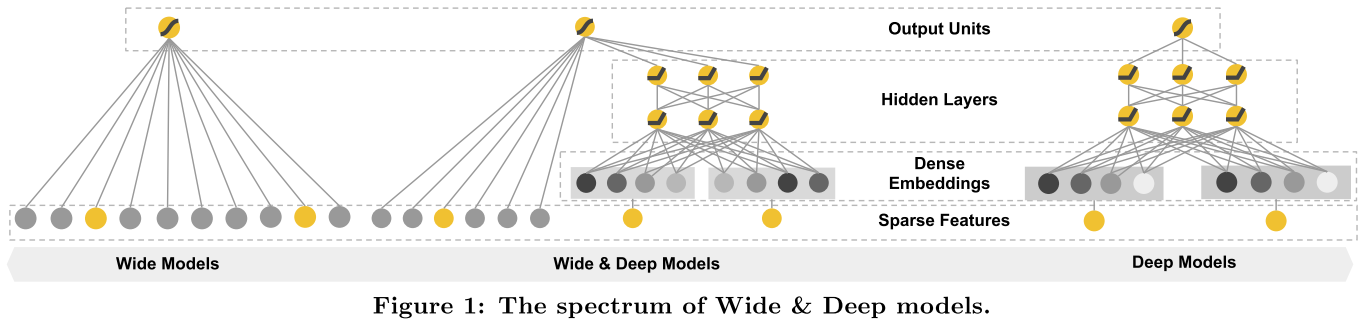推荐系统 + 深度学习 2
谷歌著名的Wide & Deep模型

# 论文背景

题目：Wide & Deep Learning for Recommender Systems
作者：Heng-Tze Cheng, Levent Koc, Jeremiah Harmsen, Tal Shaked, Tushar Chandra,
Hrishi Aradhye, Glen Anderson, Greg Corrado, Wei Chai, Mustafa Ispir, Rohan Anil, Zakaria Haque, Lichan Hong, Vihan Jain, Xiaobing Liu, Hemal Shah, Google Inc.
会议信息：DLRS ’16 September 15-15, 2016, Boston, MA, USA

谷歌引用数量：1324（截至2021年3月2日）

# 引言 INTRODUCTION

推荐系统可视为搜索排序系统，输入是用户和上下文信息的查询，输出是物品列表。类似于一般的搜索排序问题，推荐系统中的一大挑战是同时实现记忆（memorization）和泛化（generalization）。
Memorization可以宽泛地定义为学习物品或特征的共现频率并探索历史数据的相关关系。
Generalization是基于相关性的传递性并探索过去从未出现过的新的特征组合。
基于memorization的推荐系统通常更局限于和直接与用户曾有过行为的物品相关。
基于generalization的推荐系统试图增强推荐物品的多样性。

例子：如果用户安装了netflix，特征”user_installed_app=netflix”的值为1。
Memorization：通过使用稀疏特征的跨物品转换实现，例如AND(user_installed_app=netflix, impression_app=pandora”)，如果用户安装了netflix，然后显示pandora, AND的值为1。
Generalization：可以通过使用粒度更小的特性来添加，例如AND(user_installed_category=video，impression_category=music)，但是通常需要手动的特征工程。
叉积变换（cross-product transformations）的一个限制是它们不能推广到没有出现在训练数据中的查询项特征对。基于嵌入的模型，例如FM或者DNN跨域解决这个问题。但是当底层的查询项矩阵是稀疏且高阶的（例如用户具有特定的偏好或小范围的吸引力）时，很难学习查询和项的有效低维表示。在这种情况下，大多数查询项对之间应该没有交互，但密集嵌入将导致所有查询项对的预测非零，因此导致过拟合而产生不相干的推荐。具有叉积特征转换的线性模型可以用更少的参数记住这些“例外规则”。

在本文中提出了Wide&Deep模型，通过联合训练一个线性模型组件和一个神经网络组件，实现在一个模型中记忆和泛化。

• 带有嵌入和带有特征转换的线性模型的前馈神经网络联合训练的Wide&Deep学习框架，用于具有稀疏输入的通用推荐系统。
• 在谷歌Play上实施和评估，谷歌Play是一个拥有超过10亿活跃用户和超过百万应用的移动应用商店。
• 在TensorFlow中有开源代码。

# 推荐系统概述 RECOMMENDER SYSTEM OVERVIEW

推荐系统一般分为召回（Retrieval）层，排序（Ranking）层。本文在排序层使用Wide & Deep学习框架。

# 宽 & 深度学习框架 WIDE & DEEP LEARNING

## 宽度组件 The Wide Component

宽度组件是广义线性模型$y = w^Tx + b$，y是预测值，$x = [x_1, x_2, …, x_d]$是d维特征，$w = [w_1, w_2, …, w_d]$是模型参数，b是偏置项。特征集包括原始输入特征和转换后的特征。其中最重要的一种变换是叉积变换，定义为:$$\phi_k(x) = \prod_{i=1}^d{x_i}^{c_{ki}}, c_{ki}∈{0,1}$$
其中，$c_{ki}$是一个布尔变量，如果第i个特征是第k个变换$\phi_k$的一部分，则为1，否则为0。
对于二进制特征，一个叉积变换“AND(gender=female, language=en)，只有当(“gender=female” and “language=en”)时才为1。
这捕获了二元特征之间的相互作用，并为广义线性模型增加了非线性。

## 深度组件 The Deep Component

深度组件是一个前馈神经网络。对于分类特征，原始输入是特征字符串(例如 “language=en”)，这些稀疏的、高维的分类特征首先被转换成一个低维的、稠密的实值向量，通常被称为嵌入向量。嵌入的维数通常在O(10)到O(100)之间。在模型训练过程中，先对嵌入向量进行随机初始化，然后对其值进行训练，使最终损失函数最小。这些低维密集嵌入向量然后被馈入前向传递的神经网络的隐藏层。具体来说，每个隐含层计算：
$$\alpha^{l+1} = f(W^{(l)}a^{(l)}) + b^{(l)})$$

## 模型联合训练 Joint Training of Wide & Deep Model

将宽度组件和深度组件以其输出对数概率的加权和进行组合作为预测值，然后将其输入一个通用的logistic损失函数进行联合训练。
Joint training和ensemble是有区别的。
ensemble：在一个整体中，个体模型在不相互了解的情况下被单独训练，并且它们的预测只在预测时组合，而不是在训练时。由于训练是不连贯的，每个单独的模型尺寸通常需要更大(例如，有更多的特征和转换)，以达到一个集成工作的合理精度。
Joint training：联合训练通过在训练时同时考虑深度和宽度部分以及它们和的权重来同时优化所有参数。另外，宽度部分只需要用少量的叉积特征转换来弥补深度部分的弱点，而不需要一个全量的宽度模型。
采用小批量随机优化方法，将梯度从输出部分同时反向传播到模型的宽、深部分，从而实现宽、深模型的联合训练。

对于逻辑回归问题，模型的预测为：
$$P(Y=1|x) = \sigma(w^T_{wide}[x,\phi(x)]+w^T_{deep}a^{(l_f)}+b)$$
其中Y是二分类标签，$\sigma(·)$是sigmoid函数，$\phi(x)$是原始特征x的叉积变换，b是偏置项。$w_{wide}$是所有宽度模型权重的向量，$w_{deep}$是应用在最终激活$a^{(l_f)}$的权重。

# 系统实现 SYSTEM IMPLEMENTATION

## 数据产生 Data Generation

通过将一个特征值x映射到其累积分布函数P(X≤x)，将连续实值特征归一化为[0,1]，并分成$n_q$分位数。对于第i个分位数的值，规范化值为$\frac{i-1}{n_q-1}$。分位数边界i−1在数据生成时计算。

## 模型训练 Model Training

在训练过程中，输入层接收训练数据和词汇，并生成稀疏和密集特征以及标签。宽度组件包括用户安装应用和印象应用的叉积。对于模型的深层部分，每个分类特征学习一个32维的嵌入向量。将所有的嵌入与密集特征连接在一起，得到一个大约1200维的密集向量。然后将连接的矢量送入3个ReLU层，最后送入logistic输出单元。
Wide & Deep模型训练了超过5000亿个例子。每当一组新的训练数据到达时，模型就需要重新训练。然而，每次重新训练在计算上都是昂贵的，并且延迟了服务时间。为了解决这一挑战，本文实现了一个暖启动系统，该系统使用先前模型的嵌入和线性模型权值来初始化一个新的模型。

## 模型服务 Model Serving

一旦模型经过训练和验证，就把它加载到模型服务器中。对于每个请求，服务器都会从应用程序检索系统和用户特性中接收一组应用程序候选项来为每个应用程序评分。然后，应用程序从最高分到最低分进行排名，并按照这个顺序向用户展示这些应用程序。分数是通过运行一个采用Wide & Deep模型的正向推理来计算的。
为了为每个请求提供10毫秒量级的服务，使用多线程并行来优化性能，通过并行运行较小的批处理，来代替在单个批处理推理步骤中对所有候选应用程序进行评分。

# 实验 EXPERIMENT RESULTS

## App Acquisitions

本文在A/B测试框架下进行了为期3周的在线实时实验。对于对照组，随机选择1%的用户，并向他们展示由上一个版本的排名模型生成的推荐，该模型是一个高度优化的广泛性logistic回归模型，具有丰富的叉积特征转换。在实验组中，1%的用户使用了由相同的一组特征进行训练的Wide & Deep模型生成的推荐。

Wide & Deep模式使app store主登陆页面的应用获取率比对照组提高了+3.9%。结果还与另1%组仅使用具有相同特征和神经网络结构的模型的深度部分进行了比较，Wide & deep模式比deep-only模型有+1%的增益。
除了在线实验，还展示了AUC。Wide & Deep的线下AUC略高，但对线上流量的影响更显著。一个可能的原因是离线数据集中的印象和标签是固定的，而在线系统可以通过混合归纳和记忆生成新的探索性推荐，并从新的用户反应中学习。

## 服务性能 Serving Performance

面对我们的商业移动应用商店所面临的高流量，高吞吐量和低延迟的服务具有挑战性。在高峰流量时，我们的推荐服务器每秒可以获得超过1000万个应用。使用单个线程，在一次批处理中为所有候选人打分需要31毫秒。我们实现了多线程，并将每个批处理分成更小的部分，这显著地将客户端延迟减少到14毫秒（包括服务开销），如表所示。

# 相关工作 RELATED WORK

结合带叉积转换的广义线性模型与深层神经网络嵌入的灵感来自以前的工作，比如FM，通过在两个低维嵌入向量之间使用点积分解两个变量间的相互作用，将线性模型了进行推广。在本文中，通过神经网络代替点积来学习嵌入之间高度非线性的相互作用，从而扩展了模型容量。
在语言模型中，通过学习输入和输出之间的直接权值，提出了使用n元特征的递归神经网络(RNNs)和最大熵模型联合训练，以显著降低RNN的复杂性(例如，隐藏层大小)。在计算机视觉中，深度残差学习已被用于降低训练更深层次模型的难度，并通过跳过一个或多个层次的捷径连接提高准确性。
神经网络与图形模型的联合训练还被应用于基于图像的人体姿态估计。在这项工作中，探讨了前馈神经网络和线性模型的联合训练，在稀疏特征和输出单元之间直接连接，用于输入数据稀疏的通用推荐和排序问题。
在推荐系统文献中，将内容信息的深度学习与评分矩阵的协同过滤(CF)相结合来探索协同深度学习。以前的工作也曾致力于手机应用推荐系统，如AppJoy在用户的应用使用记录上使用CF。不同于之前工作中基于cf或基于内容的方法，我们在app推荐系统中，基于用户和印象数据使用Wide & Deep模型联合训练。

# 总结 CONCLUSION

宽度线性模型可以通过叉积特征变换有效地记忆稀疏特征交互，而深度神经网络可以通过低维嵌入来泛化之前未见过的特征交互。在线实验结果表明，与Wide-only和Deep-only模型相比，Wide & Deep模型有显著提高。

# 代码

https://github.com/tensorflow/tensorflow/blob/r1.11/tensorflow/python/estimator/canned/dnn_linear_combined.py

]]>
<div align="left"><br><img src="\images\Paper-wide&deep-models.png" width="300" height="180" style="float:right;"><br><br><br>　　<strong>推荐系统 + 深度学习 2</strong><br>　　<strong>谷歌著名的Wide &amp; Deep模型</strong><br><br><br> </div>
【paper】AutoRec - Autoencoders Meet Collaborative Filtering https://hubojing.github.io/2021/02/04/【Paper】AutoRec/ 2021-02-04T02:19:03.000Z 2021-02-05T08:26:07.708Z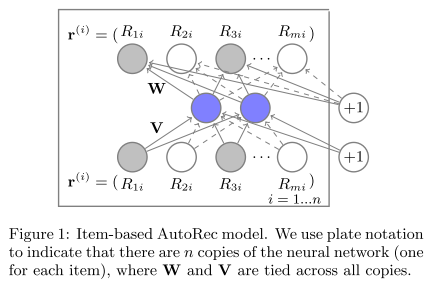推荐系统 + 深度学习 1

WWW’15

# THE AUTOREC MODEL 模型

$$min_{\theta}\sum_{r∈S}||r - h(r;\theta)||^2_2$$
$h(r;\theta)$是输入r的重构
$$h(r;\theta) = f(W · g(Vr + μ) + b)$$
f、g是激活函数。$\theta = {W, V, μ, b}$
$W∈\mathbb{R}^{d×k}$, $V∈\mathbb{R}^{k×d}$, $μ∈\mathbb{R}^k$, $b∈\mathbb{R}^d$

${r^{(i)}}^n_{i=1}$

1. 每个$r^{(i)}$通过反向传播更新和输入有关的权重得到，这在矩阵分解和RBM策略中常用。
2. 设计了学习参数正则化防止过拟合。

I-AutoRec需要估计2mk + m + k个参数。

$$\hat{R}_{ui} = (h(r^{(i)};\hat{\theta}))_u$$

$$min_{\theta}||r^{(i)}-h(r^{(i)};\theta)||^2_o + \frac{\lambda}{2}·(||W||^2_F + ||V||^2_F)$$
$||||^2_o$代表只考虑可观测评分的贡献。

${r^{(u)}}^m_{u=1}$

1. RBM-CF是基于限制玻尔兹曼机的生成概率模型，AutoRec是一个基于自动编码器的判别模型。
2. RBM-CF通过最大化似然log函数估计参数，AutoRec直接最小化RMSE。
3. 训练RBM-CF需要使用对比散度，训练AutoRec需要相对更快的基于梯度的反向传播。
4. RBM-CF只使用于离散评分，并对每个评分估计一个分散的参数集。对r个可能的评分，它使用了基于RBM的nkr或者mkr个参数用于用户（物品）。AutoRec与r无关，因此需要较少的参数。 较少的参数使AutoRec的内存占用量更少，更不容易过度拟合。

MF学习线性潜在表示，AutoRec可以通过激活函数学习非线性潜在表示。

# EXPERIMENTAL EVALUATION 实验评估

1. 和RBM对比
2. 激活函数选取对比
3. 隐藏单元k的数量
4. 基线性能对比
5. 深度扩展对Auto的帮助

# 代码

https://github.com/mesuvash/NNRec

# 总结

AutoRec是最简单的深度学习推荐系统。

]]>
<div align="left"><br><img src="\images\Paper-AutoRec-Itembased.png" width="300" height="180" style="float:right;"><br><br><br>　　<strong>推荐系统 + 深度学习 1</strong><br><br><br> </div>2020年我到底在干嘛之年终总结。

# 前言

技术博客只谈技术相关。今年年底新搭建了生活博客，所以非技术方面的总结将放在那边。不过由于前几年的年终总结关于学习方面都放在了技术博客上，因此今年依然还是把所有和学习有关的总结放在这边。

如果说今年我给自己在技术方面的评价打分的话，大概只能打个50分，反正不及格。

此为前言。

# 2020致自己完成情况

今年对自己的要求最核心的一条：自律

自己Flag立得飞起，2020年反而是自律最糟糕的一年……

这两天突然有人给我以前在知乎上的匿名发言点了个赞，我点进去一看，好家伙，我直呼好家伙，这是曾经的我？ε=(´ο｀*)))唉，现在为什么做不到了呢？

今年年中时，我曾一度警觉到这个问题，为此写了篇年中总结。可惜并没有取得好的效果，甚至感觉下半年自律做的更糟糕了。

为此我去知乎看看大神们是如何看待自律的。
有一条我认为说得在理：
自律是手段，而不是结果。

如果我把自律当做一个目标去实现它，那么可能永远做不到，就像今年这样。
自律应该是在我追逐其它目标的过程中，不自觉形成的自动行为。
可惜看到这条回答时，已经接近年终了。

所以2021年的致自己依然是

但有些想法已发生改变。

# 突然穿插的总结

一晃，研究生生涯已经走完一半了。
真快呀。去年调剂的时候，从两年研究生变成三年研究生，一开始觉得亏，一年少挣几十万啊。
刚开学时，时常期盼着早点毕业。结果现在突然就只剩一半的读研生活了，竟然开始留恋这美好的学生生活了。我想，我确实是渐渐喜欢这样的生活了吧。我甚至有了深深的感激。一是感谢爸爸的坚决。在那段时间里，我一直徘徊在再战考研和调剂中，差一点点我就选择放弃了。在做决定上，只要是我深思熟虑后，父母一直都是支持我的。但这件事上，爸爸表现得非常坚决，强烈反对我再战，坚持调剂上岸。事实证明他是对的，最近这些年，每过一年，考研大军就以50~100万的速度递增，考研也在内卷。考研这件事请，已经占用了我太多的时间，确实应该放手了。不过我一直欠考研一篇长文，每次提笔，却觉得还应该再等等。二是感谢收留我的学校。调剂那段时间太痛苦了，了解了全国所有大大小小985/211/研究所还有各种从前没听过的学校，每天收集各种调剂信息，到最后精神几近崩溃边缘。我甚至把简历都写好了，准备找工作去了。没想到就在我决定明天开始投简历的当天傍晚，收到了这所学校的复试通知。这就是缘分吧。三是感谢收留我的导师。被调剂的学生可能就和被抛弃的孩子一样，是有一种被抛弃感和无助感的，那时只希望有个人愿意收留我，而不用害怕自己又被丢掉了。幸运的是我导收留了我，并且支持我目前的研究方向。这也是缘分吧。

这一年半，大概只有两件成果还行：
1. 数模美赛F奖
2. 加权成绩班级第一

其它的都是一团浆糊……

研一上时，大部分时间被课程占据。那时很爽，毕竟我从通信跨考过来，终于成为了科班出身。坐在教室里听那些机器学习啊大数据啊什么的，身边都是计算机软工的同学，反正就是开心。那时我以为我的前途大概就是老老实实写代码刷题，看看开源项目，然后出去实习，最后能找份大厂开发的工作就很好了，所以整个研一定的目标是多参加比赛，争取拿奖。然后误打误撞入了数模的坑，真的是误打误撞，本来想多参加开发方面的比赛，但当时刚好有个数模比赛就随手参加了。之前我完全没有数模比赛经历，研究生才开始玩数模着实很晚。不过我比较幸运，第一次比赛得了一个亚太数模的三等奖，而后又得到了美赛数模的特等奖提名奖（F奖）。后来再参加数模更多关注的是自我全方位的锻炼（后来就飘了，飘了就会被打脸）。数模三个人一般是一个建模，一个写代码，一个写论文。我想每个位置都试试，现在已经全部尝试了一遍，估计就到此为止吧。数模给我的思维锻炼还有写论文的速度和技巧锻炼可能是最有用的。（如何在四天内快速地水一篇论文

另一方面，读研后，万万没想到我竟然拥有了搞学术的机会，之前我是真的以为这辈子不可能有条件再沾和学术有关的东西了。搞学术啊……突然就让人想起一些曾经不切实际的梦。还想起刚开始工作时对部门领导说，“我喜欢那些高精尖的东西”，然后把领导和老大都给整笑了的经典场景……那学术是什么啊？那不就是研究高精尖的东西最好的渠道？
所以从研一上的末尾我开始慢慢转换思路，我开始有了也许我可以试试学术的想法（危）。我开始在知乎上看有关学术的问题，装备学术资源，提高学术姿势，希望自己能科学的入门学术。然后也在那时渐渐把研究方向定了下来。（当初幸好没入CV的坑，看看现在CV就业卷成什么样……）

新想法不停地冲击我原有的规划。走学术几乎代表我就业应该选择算法岗。算法岗啊，我原来想都不会去想。但算法岗的竞争之激烈，我倒是从17年开始就有不断关注。什么一片红海啊，诸神黄昏啊，灰飞烟灭啊……内卷这个词我在知乎上已经看吐了。什么双985才过得了简历关，没有顶会顶刊、竞赛top就不要想了……

选择算法岗，仿佛就像换了一波竞争对手一样。大厂的开发似乎尚且能够一搏，但大厂的算法岗？似乎总有痴人说梦的味道。这样的纠结，持续了整个2020。

疫情在家，本来是一个弯道超车的好机会。
确实是，我就是那辆被别人超过的车……[苦涩]

本来年初的计划，我是真心认为自己可以完成的。可是有些事情真是无法预料……元月底朋友的一条朋友圈，直接把我情绪拉到崩溃边缘，我开始反思生命中的其它事情，足足失眠了四个月有余。扯远了，总之，当你专注在另一些事情时，总会搁置其它的事情。也让我明白了，所谓的平衡但平庸和专注但极致，永远只能选一个。

越努力越幸运是真的。相反，越不努力就会越不幸也是真的。

研一下在我漫长的失眠时光中稀里糊涂地过去了。终于回到了学校，研二上。怎么就研二了？我对校园的认知还停留在研一上的阶段啊。脑袋里想着，要把心思放到学习上。行动上却不是这么做的。然后一连串不幸就来了。

六级考出了历史最低分。虽然我总是美其名曰参加六级只是为了保持英语语感顺便检测下目前英语水平，每次都裸考去考试，完全不复习。看到分数后，我还能自我安慰一下说过了还行。但现实就是英语水平真的退步了，可我又做了什么提升措施呢？除了恢复了一阵子背单词以外，似乎就无计可施。

英语竞赛差2分得奖。其实做题时感觉还行，但这个结果还是让人失望。

数模国赛名落孙山。由于找队友只能找通过校内选拔的同学，我当时也比较随意，重心已不在数模比赛上，所以临时和两位不认识的校友组队。但可惜他们并非工科背景，选题时非常受限，整个比赛几乎是我数据处理，代码和论文全包，他们做一些辅助性工作。后来反思，我感觉论文本身还是可以的，但可能预测的结果与正确结果偏离较远吧。

国奖落选。和我一起拿F奖的队友，已经成功得到了国奖。评奖过程存在质疑，或许本来只有我是唯一有资格参评的人。可能我自己也觉得还不配，虽然本科已经得过，但国奖依然是我心中最神圣的存在。我还是希望，如果是我，是因为我百分百值得。但目前实力还没有到无可挑剔的地步。其实在我决定把时间分到其它方面时，我就有想到这个后果，但我还是低估了得到认可在我心中的重要性。我试图去降低学习占比，让我看起来平衡。我也高估了自己的调节能力，我以为我能潇洒地对这些评奖云淡风轻，但没想到带给我的后劲还是十足强烈。无论如何，我可能错过了读研阶段离国奖最近的一次机会。没想到有一天，我又想起这句鸡汤：最难过的事情是我本可以。

生活上的不幸，此处不展开。
亲人离世，外婆走得是那么突然，让我至今有有些不敢相信这是真的。
结束了短暂的感情。如果两个人不能相互促进，共同进步，那可能不是好的感情。

这些种种不幸一直持续到导师通知我坐飞机参加会议。我当时甚至觉得这阵子我实在是太惨了，以至于我真的认为我坐飞机都会掉下来。

我想，也许越努力越幸运是真的。越不努力也可能真的越不幸。
我开始重新整理自己，把有些过多陷入感性的自己再次拉回来。“我试图去降低学习占比”，我为什么要降低学习占比？？？我才发现追求平衡这种事情多少有些可笑，读研不代表真正地上岸，如果读研了就降低学习占比，那是否对得起之前那些年舍弃那么多去考研？我想我已经受到了足够多的警示了，这些事情无一不在告诉我，学生不始终把学习放第一位，等于自我毁灭。

幸运的是，飞机没有掉下来。从那刻起，我感觉自己如获新生。我想，我可能要开始转运了。
首先是教资成绩下来，预想能过一门就值了报名费，没想到三过二，超过预期。然后是软考，只复习几天竟然有惊无险地过了。爸爸身体抱恙，但医生说无大碍。

划水划到最后总会还的。当我重新整理我的代码，搜集的论文，我的博客，都无一不在说，你慢了。我终于开始为前途担忧，为进度焦虑，为找工作考虑，开始新一轮的失眠。

# 去年年初立下的Flag完成度情况

2020——新的启航

## 科研方面

1. 多看论文，完成导师任务。
2. 多了解工业届具体应用情况，了解就业市场需求。
3. 对推荐系统，乃至NLP，以及相关传统机器学习、深度学习的代码实操，技术路线搭建。
1. 不达标。进度慢了。
2. 基本达标。还行，经常有关注。
3. 不达标。代码方面还是非常不够，需要加强。

完成度：50%

## 技术方面

1. Leetcode一周三题，一年156题。
2. 纸质书五本。
3. 至少细致分析一个开源项目代码。
4. Java学习。
1. 不达标。远远不够，才做20题左右。
2. 基本达标。勉强够吧，如果把应考的书也算上的话。
3. 不达标。细致分析达不到。
4. 不达标。后半年几乎停滞。

完成度：10%

## 日常学习方面

1. 微信读书一周2h，一年104h。（截止今日：73h11min）
2. 纸质书（非技术类）两月一本，一年六本。今年读书重点依然在推理、历史类，可辅看经济入门书。
3. 英语电台更新大于100期。
1. 达标。今年读了109h（来自官方报告）。（截至2020年12月31日：181h10min）
2. 不达标。纸质书非技术类一本没读……
3. 不达标。只更新了58期。

完成度： 60%

## 健身方面

1. 不熬夜。熬夜则自动领取加读论文一篇的奖励，需在博客上有记录。
2. Keep 3000min。一周至少三次Keep，运动量大于30min，一年需运动4680min（然而截止2019年底也才2530min），所以折中一下。
1. 不达标。今年熬夜是前二十几年最凶猛的，不仅熬夜，还失眠……补论文估算了一下至少得补100篇……
2. 不达标。856min。

完成度：0%

这样一看，给自己总评打50分似乎打高了……

# 2021年的目标

今年突然意识到，这个年纪还能有人跟你说“要好好学习哦”，是多么幸福的事情。

立Flag一时爽，可是做不到的Flag就是打脸现场。比如现在，脸已经肿了……
2021年不再立一大堆花里胡哨的目标了。
2021年的目标，ONLY ONE：
闭关学习，拿到大厂Offer！

少说话，多做事。Talk is cheap, show me the code.

]]>
<div align="left"><br><img src="\images\打脸现场——2020年终总结.jpg" width="300" height="180" style="float:right;"><br><br><br>　　<strong>2020年我到底在干嘛之年终总结。</strong><br><br><br> </div>
【Paper】Deep Neural Networks for YouTube Recommendations https://hubojing.github.io/2020/12/15/【Paper】Deep Neural Networks for YouTube Recommendations/ 2020-12-15T06:24:54.000Z 2020-12-16T13:56:05.000Z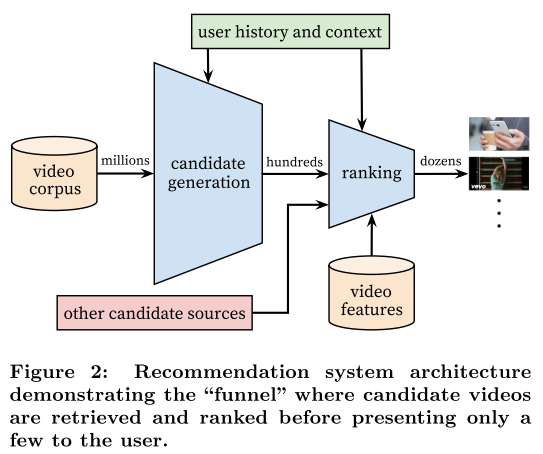# 论文背景

RecSys’16
谷歌学术引用次数为1201（截至2020年12月15日）

作者：Paul Covington, Jay Adams, Emre Sargin
Mountain View, CA

关键词：recommender system; deep learning; scalability
PDF

# Introduction 引言

• Scale 大规模
• Freshness 新鲜度
• Noise 噪声-数据往往是隐式反馈

# System Overview 系统概述

系统由两部分组成：候选生成部分和排序部分。
候选生成部分输入是用户历史活动事件，使用协同过滤从大数据集中输出小子集。
排序部分根据目标函数对每个视频精排打分，最高分视频推荐给用户。

优点：从大数据集中选出的小数据集依然具有个性化特征，并且候选生成部分可以添加别的数据源。

评价指标：精确率、召回率、排序损失等
最后通过A/B测试在线实验（A/B测试结果不总是和离线实验结果相一致）。

# Candidate Generation 候选生成

这种方法可以看作矩阵分解的非线性推广，它早期的神经网络迭代只嵌入用户过去观看记录来模拟这种因子分解行为。

## Recommendation as Classification 作为分类推荐

推荐视为极端多分类问题，在时间t时，结合用户U和上下文C，从数亿的数据库V中选择一个视频i记为特定的视频$w_t$。
$$P(w_t = i|U,C) = \frac{e^{v_iu}}{\sum_{j∈V}e^{v_ju}}$$
其中$u∈\mathbb{R}^N$代表了用户高维嵌入（embedding），上下文数据对和$V_j∈\mathbb{R}^N$代表了每个候选视频的嵌入（embedding）。
在这样的设定中，一个嵌入就从稀疏实体转为了稠密向量（$\mathbb{R}^N$）。

深度神经网络的任务是学习用户嵌入u，作为用户历史记录和上下文的函数，这有助于使用softmax分类器从海量视频中进行识别。

### Effcient Extreme Multiclass 高效极端多分类

为了有效地训练这样一个拥有数百万分类的模型，需要依赖于一种技术，从背景分布(“候选抽样”)中抽取负类，然后通过重要性加权对该抽样进行修正。对于每一个实例，真标签和抽样的负样本的交叉熵损失都最小。
由于服务时不需要softmax输出层校准可能性（？有些不解），评分问题简化为在点积空间的最近邻搜索，在这个空间中，通用库可以使用。A/B结果对最近邻搜索算法的选择不是特别敏感。

## Model Architecture 模型架构

学习每个视频的高维嵌入到固定词汇中，并将这些嵌入输入到前馈神经网络中。用户的观看历史记录由稀疏视频id的可变长度序列表示，该序列通过嵌入映射到稠密向量表示。该网络需要固定规模的密集输入，并简单地平均在不同策略中表现最好的嵌入。通过梯度下降反向传播更新，嵌入与所有其他模型参数联合学习。特征被连接成一个宽的第一层，然后是几层全连接的线性单元(ReLU)。如下图所示。

## Heterogeneous Signals 混合信号

使用深度神经网络作为矩阵分解的推广的一个关键优点是任意连续和分类特征可以很容易地添加到模型中。

### “Example Age” Feature “示例年龄”特征

用户往往喜欢新鲜的内容。除了简单地推荐用户想看的新视频的一级效应，还有一个重要的二级现象，即引导和传播病毒式内容。视频受欢迎程度是会变化的，但推荐系统反映的是几周内的平均观看可能性。为了纠正这一点，在训练期间将训练示例的年龄作为一个特征输入。在服务时间，这个特征被设置为零(或者稍微负一点)，以反映模型正在训练窗口的最后进行预测。

## Label and Context Selection 标签和上下文选择

替代学习问题的选择对A/B测试的性能有很大的影响，但很难用离线实验来衡量。
必须非常小心地对分类器隐瞒信息，以防止模型利用站点的结构和过度拟合代理问题。
预测用户下一个观看的性能要比预测随机留出的观看好得多。许多协同过滤系统隐式地选择标签和上下文，方法是取出一个随机的物品，并从用户历史记录中的其他物品中预测它。这泄露了未来信息并忽视了非对称消费模式。相反，本文“回滚”用户的历史记录，通过选择一个随机的观看记录，并且只输入用户留出的有标签的观看记录之前采取的动作。

## Experiments with Features and Depth 特征和深度实验

添加特征和深度可以显著改善保持数据的精度，如图6所示。在这些实验中，一个包含100万个视频和100万个搜索令牌的词汇表中嵌入了256个浮点数，每个浮点数的最大包尺寸为50个最近的观看和50个最近的搜索。softmax层在相同的1M视频类上输出多项分布，维数为256(可以认为是单独的输出视频嵌入)。网络结构遵循一个常见的“塔”模式，其中网络的底部是最宽的，每个后续隐藏层的单位数量减半。

# Ranking 排序

使用与候选生成结构相似的深度神经网络，使用逻辑回归为每个视频印象分配一个独立的分数。如图所示。然后，视频列表将根据这个分数进行排序并返回给用户。最终排名目标是根据实时A/B测试结果不断调整，但通常是每个印象的预期观看时间的简单函数。通过点击率排名通常会促进欺骗性视频，用户没有完成(“点击诱饵”)，而观看时间能够更好地捕捉用户粘性。

## Feature Representation 特征表示

用的类别或特征在基数上差别很大——有些是二进制的（例如用户是否登录），而其他有数百万个可能的值（例如用户的最后一次搜索查询）。根据功能是仅贡献单个值(“univalent”)还是一组值(“multivalent”)，进一步划分功能。
univalent例子：被评分的视频ID，multivalent例子：用户最近观看的N个视频ID的bag。还根据特性是描述物品的属性（”impression”）还是描述用户/上下文的属性（”query”）对特性进行分类。查询特征为每个请求计算一次，而印象特征为每个物品项计算一次。

### Feature Engineering 特征工程

描述过去视频输入频率的特性对于在推荐中引入“churn”(连续的请求不返回相同的列表)也是至关重要的。如果一个用户最近被推荐了一个视频，但没有看过它，那么模型自然会在下一次加载页面时降低这种印象。

### Embedding Categorical Features 嵌入分类特征

类似于候选生成，使用嵌入将稀疏分类特征映射到适合于神经网络的稠密表示。
同一ID空间中的分类特性也共享底层的嵌入。共享嵌入对于提高泛化性能、加快训练速度和减少内存需求具有重要意义。

### Normalizing Continuous Features 归一化连续特征

神经网络对其输入的尺度和分布非常敏感，而其他方法，如决策树的集合，对单个特征的尺度是不变的。
对于连续特征x，其分布为f，使用公式$\tilde{x} = \int_{-\infty}^xdf$进行归一化操作到[0,1)。除了该方法，也使用了$\tilde{x}^2$和$\sqrt{\tilde{x}}$。

## Modeling Expected Watch Time 预期观看时间建模

预期观看时间使用加权逻辑回归方法。
模型在交叉熵损失下用逻辑回归进行训练。积极的（点击）印象是由视频上观察的观看时间加权的。负面（未点击的）印象全部获得单位权重。
逻辑回归学到的概率为$\frac{\sum{T_i}}{N-k}$，N是训练集数量，k是正例数量，$T_i$是第i个印象的观看时间。
最终本文使用指数函数$e^x$作为最后激活函数来产生预估概率。

## Experiments with Hidden Layers隐藏层实验

这些结果表明，增加隐藏层的宽度可以改善结果，同时也可以增加它们的深度。但是，需要权衡的是推荐所需的服务器CPU时间。

# Conclusions 总结

通过捕捉不对称的协同观看行为和防止未来信息泄漏，对未来观看进行分类，可以很好地执行实时指标。从分类器中截取不同信号对于取得良好的结果也是至关重要的——否则模型会过度拟合代理问题而不能很好地转移到主页。
使用训练样例的年龄作为输入特征，消除了对过去的固有偏见，并允许模型表示流行视频的时间依赖性行为。这提高了离线保持精度结果，并在A/B测试中显著增加了观看最近上传的视频的时间。
排序是一个比较经典的机器学习问题，然而本文深度学习方法在观看时间预测方面优于以往的线性和基于树的方法。推荐系统尤其受益于描述用户关于物品的历史行为的特定特征。深度神经网络需要分类特征和连续特征的特殊表示，分别用嵌入和分位数规范化进行变换。层的深度显示有效地模拟了非线性交互之间的数百个特征。
逻辑回归通过加权训练样例修正了正例，统一了负例，使之能学习预期观看时间的概率。与直接预测点击率相比，该方法在观看时间加权排序评价指标上表现得更好。

]]>
【Paper】Factorization Machines https://hubojing.github.io/2020/12/09/【Paper】Factorization Machines/ 2020-12-09T03:18:31.000Z 2020-12-14T12:08:40.000Z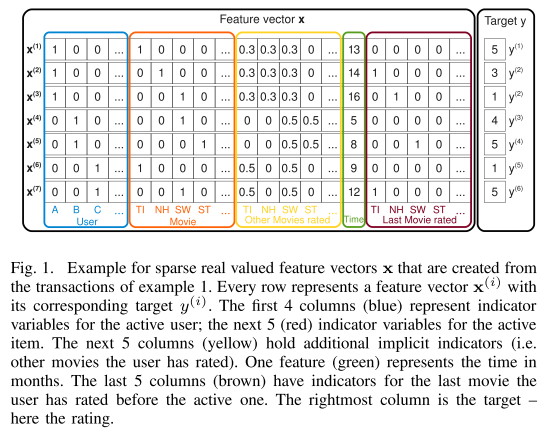FM算法原文。

# 论文背景

2010 IEEE International Conference on Data Mining
Steffen Rendle
Department of Reasoning for Intelligence
The Institute of Scientific and Industrial Research Osaka University, Japan

论文关键词：factorization machine; sparse data; tensor factorization; support vector machine
PDF

# Introduction 引言

FM优点：
1. FM能在很稀疏的数据上进行参数估计，但SVM不行。
2. FM是线性复杂度，不需要类似于SVM中的支持向量。
3. FM是通用预测方法，适用于任何特征向量。其它的因子分解方法都受限于特定的输入。（对比biased MF, SVD++, PITF和FPMC）

# Factorization machines(FM) 因子分解机

## Factorization machine model 因子分解机模型

degree d = 2
$$\hat{y}(x):=w_0 + \sum_{i=1}^nw_ix_i + \sum_{i=1}^n\sum_{j=i+1}^n<v_i,v_j>x_ix_j$$
其中$w_0∈R$，$w∈R^n$,$V∈R^{n×k}$，<·,·>表示点乘。
$$<v_i,v_j>:=\sum_{f=1}^kv_{i,f}·v_{j,f}$$
V中的$v_i$描述k个因素中的第i个变量。$Ks∈N_0^+$是定义因子分解的维度的超参数。
2-way FM捕捉所有变量的单个和成对交互：
$w_0$是全局偏置，$w_i$模拟了第i个变量的程度。
$\hat{w}_{i,j}:=<v_i, v_j>$模拟了第i和第j个变量间的交互。这个在高阶交互时（d > 2）高质量估计的关键。
时间复杂度O($kn^2$)，因为所有的交互对都要被计算。但可以变形使之化为O(kn)。

## Factorization machines as predictors FM作为预测器

可用于回归、二分类和排序问题。

## Learning factorization machines FM学习策略

使用随机梯度下降（SGD）来学习。

## d-way factorization machine 多维FM

同时2-way FM可以拓展为d-way。

## Summary 总结

FM优点：
1. 在高稀疏下可估计特征交互，尤其是不可观测的交互。
2. 预测和学习的时间复杂度是线性的。使SGD优化可行，并允许多种损失函数优化。

# FMs vs. SVMs 因子分解机对比支持向量机

## SVM model 支持向量机模型

SVM等式$$\hat{y}(x) = <\Phi(x), w>$$
其中$\Phi$是从空间$R^n$到F的映射。$\Phi$和下式的核相关：
$$K:R^n × R^n → R, K(x, z) = <\Phi(x), \Phi(z)>$$
1) 线性核
$K_l(x, z) = 1 + <x, z>$，对应$\Phi(x) := (1, x_1, … , x_n)$
SVM等式为
$$\hat{y}(x) = w_0 + \sum^n_{i=1}w_ix_i, w_0∈R, w∈R^n$$
这等价于FM中d = 1的情况。

2) 多项式核
多项式核使SVM能模拟变量间的高阶交互。
$K(x, z) := (<x, z> + 1)^d$，对于d = 2有
$\Phi(x) := (1, \sqrt{2}x_1, … , \sqrt{2}x_n, x_1^2, … , x_n^2, \sqrt{2} x_1x_2, … , \sqrt{2}x_1x_n, \sqrt{2}x_2x_3, … , \sqrt{2}x_{n-1}x_n$
SVM等式为
$$\hat{y}(x) = w_0 + \sqrt{2}\sum^n_{i=1}w_ix_i + \sum^n_{i = 1}w_{i,i}^{(2)}x_i^2 + \sqrt{2}\sum^n_{i=1}\sum^n_{j=i+1}w_{i,j}^{(2)}x_ix_j$$
其中$w_0∈R, w∈R^n, w^(2)∈R^{n×n}$。
d = 2时，FM和SVM的区别在于SVM中$w_{i,j}$是完全独立的，而FM中参数是因子分解的，所以$<v_i, v_j>$依赖于彼此。

## Parameter Estimation Under Sparsity 在稀疏情况下的参数估计

举例：使用协同过滤（上图中蓝色和红色部分数据）。
1) 线性SVM
$$\hat{y}(x) = w_0 + w_u + w_i$$
只有j = u 或 j = i时$x_j$ = 1，即只有用户和物品偏好被选中时才有用。由于模型简单，在稀疏情况也能进不错的参数估计，但预测质量低。
2) 多项式SVM
$$\hat{y}(x) = w_0 + \sqrt{2}(w_u + w_i) + w_{u,u}^{(2)} + w_{i,i}^{(2)} + \sqrt{2}w_{u,i}^{(2)}$$
$w_u$和$w_{u,u}^{(2)}$是一样的。该等式除了一个额外的$w_{u,i}$，等价于线性SVM。在训练集中，对于每一个$w_{u,i}$最多只有一条记录，在测试集中通常没有。因此，2-way的SVM效果也不比线性SVM好。

## Summary 总结

1) SVM需要直接观测交互数据，但稀疏数据集经常没有。FM参数可以在系数情况下进行不错的参数估计。
2) FM可以一开始就直接学习，但非线性SVM需要成对学习。
3) FM是独立于训练集的，SVM的预测是基于部分训练数据的。

# FMs VS. Other Factorization Models 其它因子分解方法对比

改写FM公式形式，分别与Matrix and Tensor Factorization矩阵和张量分解、SVD++、PITF for Tag Recommendation、Factorized Personalized Markov Chains(FPMC)方法对比，FM改写后性能与这些方法实现效果类似。

## Summary 总结

1) 标准因子分解模型（比如PARAFAC或者MF）不像FM一样是通用预测模型。
2) 修改特征提取部分，FM可以模拟在特定任务下的成功模型（比如MF,PARAFAC,SVD++,PITF,FPMC)。

# Conclusion and Future Work 总结和展望

与SVM对比，
1) 在数据稀疏情况下，FM可以进行参数估计。
2) 模型时间复杂度是线性的，并且只依赖于模型参数。
3) 从最开始就能直接优化。

]]>
<div align="left"><br><img src="\images\Paper-FM-feature_x.png" width="300" height="180" style="float:right;"><br><br><br>　　<strong>FM算法原文。</strong><br><br><br> </div>
【Paper】Matrix Factorization Techniques for Recommender Systems https://hubojing.github.io/2020/12/07/【Paper】Matrix Factorization Techniques for Recommender Systems/ 2020-12-07T07:12:57.000Z 2020-12-09T03:23:00.422Z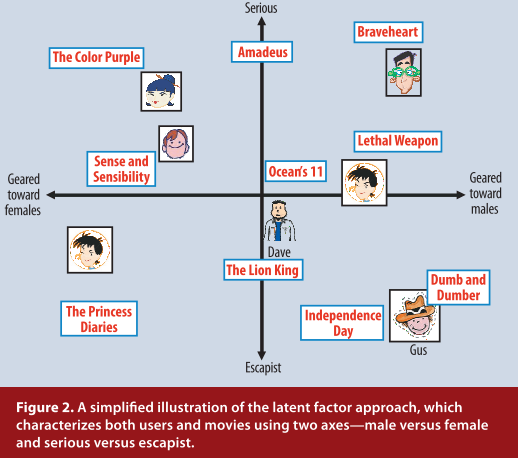矩阵分解算法原文。

# 论文背景

2009年发表于IEEE旗下的Computer期刊。
谷歌学术引用数为7954（截至2020年12月7日）。
作者：Yehuda Koren, Yahoo Research
Robert Bell and Chris Volinsky, AT&T Labs—Research
DOI: 10.1109/MC.2009.263
PDF

# Recommender System Stratrgies 推荐系统策略

两种策略：content filtering approach和collaborative filtering
前者需要收集外部信息，但这不容易得到。后者聚焦于用户过去的行为，相比前者精确度更高，但它有冷启动问题。相反，在冷启动问题方面，前者更优越。

协同过滤又分为neighborhood methods和latent factor models。
基于领域的策略又可分为基于用户和基于物品。
基于物品的策略基于同一个用户对相邻物品的评分对用户偏好进行评估。同一个用户给一件物品的相邻物品会打相似的评分。
基于用户的方法鉴定相似的用户，他们可以互相补充对方的评分信息。

LFM隐语义模型意思是把特征刻画分为k个维度。如图，将刻画特征设为性别和题材类别（serious/escapist），形成一个坐标空间，每个用户和物品都在这个空间中，如果在坐标系中距离越近则说明越相似。

# Matrix Factorization Methods 矩阵分解策略

一些最成功的隐语义模型是基于矩阵分解实现的。
矩阵分解通过从评分矩阵推断出的因子向量来刻画物品和用户。
优势：当显式反馈无法得到时，可以添加其它信息（比如使用隐式反馈推断用户偏好）。

# A Basic Matrix Factorization Model 基本矩阵分解模型

矩阵分解模型将用户和物品映射到维度f的联合潜在因素空间，用户-物品交互在空间中使用内积建模。
每一个item的向量设为$q_i$，每一个用户的向量设为$p_u$。$q_i$表示item拥有的积极或消极的因子，$p_u$表示用户拥有的积极或消极的因子。两者内积可以捕捉用户u和item i之间的交互，即用户在这个item上的整体兴趣。设评分为$r_{ui}$，
$$\hat{r}_{ui} = {q_i}^T{p_u}$$
这个模型和SVD（singular value decomposition）很接近。但在协同过滤中使用SVD要用到用户-物品评分矩阵，这个矩阵稀疏性太大。如果数据不完整，SVD不能被确定。并且用少数数据尝试容易过拟合。
早期系统都在填补缺失评分和使评分矩阵更稠密上下功夫。但是这个运算很昂贵。因此，更多的工作聚焦在直接观察现有评分数据，并通过正则化来避免过拟合。为了学习${p_u}$和${q_i}$，使用下述公式
$$min_{q*,p*}\sum_{(u,i)∈k}({r_ui} - {p_u}^Tq_i)^2 + \lambda(||p_u||^2 + ||q_i||^2)$$
其中，k是(u,i)对的集合。${r_{ui}}$是未知的（训练集）。

# Learning Algorithms 学习算法

使上述式子最小化的两种方法是随机梯度下降和交替最小二乘法。

计算error
$$\begin{equation}{e_{ui}}\overset{def}{=} {r_{ui}} - {q_i}^T{p_u}\end{equation}$$

调参
$${q_i} \leftarrow q_i + \gamma·({e_ui}·{p_u} - \lambda · {q_i})$$
$${p_u} \leftarrow p_u + \gamma·({e_ui}·{q_i} - \lambda · {p_u})$$

该方法运行速度较快。不过在有些场景下，使用ALS优化更好。

## Alternating least squares 交替最小二乘法

一般随机梯度下降比ALS简单且快。但ALS适用于两个场景，一是系统可以并行化。ALS可独立计算${q_i}$和${p_u}$。二是隐式数据情况下使用。

每个用户评价严格度不同，因此引入偏置项。
$${b_{ui}} = μ + {b_i} + {b_u}$$
加了偏置项后，公式改为
$$\hat{r}_{ui} = μ + {b_i} + {b_u} + {q_i}^T{p_u}$$
四部分：全局均值、item偏置、user偏置和user-item交互。
系统通过最小化平方误差函数来学习：
$$min_{p*,q*,b*}\sum_{(u,i)∈k}({r_ui} - μ - {b_u}- {b_i}-{p_u}^Tq_i)^2 + \lambda(||p_u||^2 + ||q_i||^2 + b_u^2 + b_i^2)$$

$$\hat{r}_{ui} = μ + {b_i} + {b_u} + {q_i}^T[{p_u} + |N(u)|^{-0.5} \sum_{i∈N(u)}{x_i} + \sum_{a∈A(u)}{y_a}]$$
N(u)指用户u有过隐式反馈的若干个item集合。x是和item i相关的因素。$\sum_{i∈N(u)}{x_i}$表示一个用户对N(u)中的若干item的偏好刻画向量。系数代表规范化。
用户属性用A(u)表示，每一个用户的每一个属性对应的因素向量用$y_a$表示。$\sum_{a∈A(u)}{y_a}表示每个用户的属性集。 # Temporal dynamics 时空动态 用户兴趣会发生兴趣漂移，即随着时间而变化。 $$\hat{r}_{ui}(t) = μ + b_i(t) + b_u(t) + q_i^Tp_u(t)$$$b_i(t)$表示物品随时间变化的流行程度，$b_u(t)$表示用户评分随时间变化的严格程度，$p_u(t)$表示用户偏好随时间变化的改变程度。物品是静态的，所以$q_i$也是静态的。 # Inputs with varying confidence levels 各种信任级别的输入 不是每一条评分数据的权重和信任都是一样的。为了给不同的评分以不同的信任程度，修改损失函数为 $$min_{p*,q*,b*}\sum_{(u,i)∈k}c_{ui}({r_ui} - μ - {b_u}- {b_i}-{p_u}^Tq_i)^2 + \lambda(||p_u||^2 + ||q_i||^2 + b_u^2 + b_i^2)$$$c_{ui}为评分的信任程度。 # Netflix prize competition Netflix大奖竞赛 使用矩阵分解的方法取得了第一名的成绩。 加的因素越多，精确度越高。 # 阅后总结 本文主要介绍了矩阵分解的具体算法。 ]]> <div align="left"><br><img src="\images\Paper-MF-LFM.png" width="300" height="180" style="float:right;"><br><br><br> <strong>矩阵分解算法原文。</strong><br><br><br> </div> 【Paper】Amazon.com Recommendations Item-to-Item Collaborative Filtering https://hubojing.github.io/2020/12/02/【Paper】Amazon.com Recommendations Item-to-Item Collaborative Filtering/ 2020-12-02T06:40:23.000Z 2020-12-03T07:45:24.264ZItemCF原文。 # 论文背景 22 January 2003 谷歌学术被引用次数：6769（截至2020年12月2日） 期刊：IEEE Internet Computing DOI: 10.1109/MIC.2003.1167344 作者：Greg Linden,Brent Smith,and Jeremy York • Amazon.com PDF # 引言 电子商务推荐算法面临的挑战： • 一家大型零售商可能拥有大量数据、数千万客户和数百万不同的物品 • 要求在不超过半秒钟的时间内实时返回结果集，同时能产生高质量的推荐 • 新客户通常只有非常有限的信息，仅有少量购买信息和产品评分 • 基于成千上万次的购买和评分，老用户可能拥有大量信息 • 客户数据是不稳定的：每次交互都提供有价值的客户数据，算法必须对新信息立即做出响应 # Recommendation Algorithms 推荐算法 三种常规方法： 传统协同过滤、聚类模型和基于搜索的策略 traditional collaborative filtering, cluster models, and search-based methods ## Traditional Collaborative Filtering 传统协同过滤 传统协同过滤，这里指基于用户的协同过滤方法。 相似度计算：使用该方法时间复杂度最差为O(MN)，由于用户向量稀疏，时间复杂度更接近于O(M + N)。（大部分用户只涉及很少的物品，每个用户是O(M)，但一小部分用户买了很多物品，需要O(N)时间。） M为用户数，N为物品数 解决方法是降低数据规模。 通过随机采样用户或者忽视只有少数购买记录的用户，来减少M。 通过忽视非常流行或不流行的物品，来减少N。 还可以通过产品类别或或客观分类来分割物品成为小向量来减少物品数量。 维度减少，比如聚类或主成分分析可以减少M或N的大量因素。 但是上述方法会降低推荐质量。 ## Cluster Models 聚类模型 该算法的目标是将用户分配到包含最相似用户的簇。然后，它使用该簇中用户的购买和评级信息来推荐。 一旦该算法生成了簇，它就计算用户与每个簇的向量的相似性，然后选择具有最大相似性的簇，并相应地对用户进行分类。一些算法将用户分为多个簇，并描述每个关系的强度。 优点：比上述协同过滤具有更好的在线可扩展性和性能，复杂且昂贵的聚类计算是离线运行的。 缺点：推荐效果差。通过使用大量细粒度的聚类来提高质量是可能的，但是线上用户细分聚类变得几乎和使用协同过滤寻找相似客户一样昂贵。 ## Search-Based Methods 基于搜索的策略 基于搜索或基于内容的方法将推荐问题视为对相关项目的搜索。对于给定用户购买和评级信息的物品，该算法构建搜索查询来查找由相同作者、艺术家或导演或具有相似关键字或主题的其他流行项目。例如，如果一位顾客购买了《教父》系列影碟，系统可能会推荐其他犯罪题材的电影、其他由影星马龙·白兰度主演的电影或其他由弗朗西斯·福特·科波拉执导的电影。 优点：用户购买和评分记录少时，性能不错。 缺点：推荐质量低，推荐的物品太一般（general)或太狭窄（narrow）。 # Item-to-Item Collaborative Filtering 基于物品的协同过滤 ## How It Works 如何工作 Item-to-item collaborative filtering matches each of the user’s purchased and rated items to similar items, then combines those similar items into a recommendation list. 基于物品的协同过滤将用户购买的和评分的每个物品与相似的物品进行匹配，然后将这些相似的物品组合成推荐列表。 为了确定给定物品的最相似匹配，该算法通过查找用户倾向于一起购买的物品来构建相似物品表。可通过遍历所有物品并为每一对计算相似性来构建物品矩阵。然而，许多产品对没有共同的用户，因此这种方法在处理时间和内存使用方面效率低下。以下迭代算法通过计算单个物品和所有相关物品之间的相似性提供了更好的措施： 伪代码 计算两个物品之间的相似性有多种方法，一种常见的方法是使用前面描述的余弦度量，其中每个向量对应于一个物品而不是一个客户，并且向量的多维度对应于已经购买该物品的用户。相似物品表的这种离线计算非常耗时，最糟糕的情况是O(N^2M)。然而，在实践中，它更接近于零，因为大多数客户只有很少的购买记录。对购买畅销物品的用户进行采样会进一步减少运行时间，而质量几乎没有下降。 给定相似物品表，该算法找到与每个用户的购买和评分相似的物品，汇总这些物品，然后推荐最受欢迎或相关的物品。这种计算非常快速，仅取决于用户购买或评分的物品数量。 ## Scalability: A Comparison 可扩展性 Amazon.com有超过2900万的顾客和数百万的商品目录。对于非常大的数据集，可扩展的推荐算法必须离线执行最昂贵的计算。 现有算法的缺点： • 传统的协同过滤很少或没有离线计算，其在线计算随着用户和物品目录的数量而变化。该算法在大数据集上是不切实际的，除非它使用降维、采样或分区——所有这些都会降低推荐质量。 • 聚类模型可以离线执行大部分计算，但是推荐质量相对较差。为了改善这一点，可以增加细分簇的数量，但这使得在线用户细分分类变得昂贵。 • 基于搜索的模型离线构建关键字、类别和作者索引，但无法提供有趣的、有针对性的主题推荐。对于有大量购买和评分的用户来说，它们的可扩展性也很小。 可扩展性和性能的关键是它离线创建昂贵的相似物品表。该算法的在线组件——为用户的购买和评分物品查找相似的物品——独立于物品目录大小或客户总数，它只取决于用户购买或评分物品。因此，即使对于非常大的数据集，该算法也是快速的。因为该算法推荐高度相关的相似物品，所以推荐质量很好。与传统的协作过滤不同，该算法在有限的用户数据下也表现良好，仅基于两三个物品就能产生高质量的推荐。 # Conclusion 总结 主要是介绍了基于物品的协同过滤思想。 耗时昂贵的操作放在线下离线进行，使线上达到实时要求。 ]]> <div align="left"><br><img src="\images\假装有图片.jpg" width="300" height="180" style="float:right;"><br><br><br> <strong>ItemCF原文。</strong><br><br><br> </div> 关于RSS订阅 https://hubojing.github.io/2020/12/02/关于RSS订阅/ 2020-12-02T01:57:14.000Z 2020-12-02T02:35:09.510Z感谢订阅博客的各位 热爱可抵岁月漫长 昨天有位朋友私信我说要RSS订阅我的博客，突然就想起这些年写博，遇到好些朋友都这样说。 然而我自己却从来没有订阅过自己的博客（滑稽表情：大概是太烂看不下去吧 每次魔改博客后，文章tag什么的都改过好多次，甚至有的文章内容都更新过好多次。突然想看看RSS推送我的博客会是什么样子，是否会对关注我的朋友造成困扰。 上次用RSS聚合软件还是好多年前了，那时用的什么我已经不太记得了，可能是深蓝阅读？ 在知乎成为我的搜索引擎后，我熟练地打开它就是一顿暴搜“2020年还有什么好用的RSS APP”？ 答案是：没有。 这就离谱有木有？ 推荐系统把人都给控制得舒舒服服的，人们都不愿意自己主动订阅了？ 幸好…… 幸好我就是研究推荐系统的（再次滑稽 那么这篇文章到底想说什么呢？ 就是…… 我最后选择了轻芒杂志作为我的RSS阅读器。 不过这个破软件还得要开会员才能订阅RSS。 好在我去网上随便搜了个邀请码获得了会员权限。（免费的快乐） 然后我也有了自己的邀请码。 你们需要的话，输入：靖待小太阳 就可以获取永久会员啦~ ……写的像软文一样，这公司应该给我打钱。 所以，有什么更好的RSS软件吗？ 主要是稳定，别用一阵子就垮了，资料白收藏了。然后是免费！穷人的字典里充满了免费二字。其它的功能，就随缘吧，2020年了，似乎都没人用RSS了，还要什么自行车。 最后，感谢订阅博客的朋友们，你们的点评和关注助我前行。博客会一直写下去，热爱可抵岁月漫长。 ]]> <div align="left"><br><img src="/images/关于RSS订阅_cover.jpg" width="300" height="180" style="float:right;"><br><br><br> <strong>感谢订阅博客的各位</strong><br> <strong>热爱可抵岁月漫长</strong><br><br><br> </div> 深度学习常用数学知识 https://hubojing.github.io/2020/08/15/深度学习数学知识/ 2020-08-15T03:00:19.000Z 2020-08-15T03:00:19.000Z编辑这些公式给我累si了 同样适合考研狗收藏 非常欢迎纠错 # 高等数学 ## 导数定义 导数和微分的概念f’({x_0})=\underset{\Delta x\to 0}{\mathop{\lim }}\,\frac{f({x_0}+\Delta x)-f({x_0})}{\Delta x}$（1） 或者：$f’({x_0})=\underset{x\to {x_0}}{\mathop{\lim }}\,\frac{f(x)-f({x_0})}{x-{x_0}}$（2） ## 左右导数导数的几何意义和物理意义 函数$f(x)$在$x_0$处的左、右导数分别定义为： 左导数：$f’_{-}(x_0)=\underset{\Delta x\to {0^{-}}}{\mathop{\lim }}\,\frac{f({x_0}+\Delta x)-f({x_0})}{\Delta x}=\underset{x\to x_{0}^{-}}{\mathop{\lim }}\,\frac{f(x)-f({x_0})}{x-{x_0}},(x={x_0}+\Delta x)$右导数：$f’_{+}(x_0)=\underset{\Delta x\to {0^{+}}}{\mathop{\lim }}\,\frac{f({x_0}+\Delta x)-f({x_0})}{\Delta x}=\underset{x\to x_0^{+}}{\mathop{\lim }}\,\frac{f(x)-f({x_0})}{x-{x_0}}$## 函数的可导性与连续性之间的关系 Th1: 函数$f(x)$在$x_0$处可微$\Leftrightarrow f(x)$在$x_0$处可导 Th2: 若函数在点$x_0$处可导，则$y=f(x)$在点$x_0$处连续，反之则不成立。即函数连续不一定可导。 Th3:$f’(x_0)$存在$\Leftrightarrow f’_{-}(x_0)=f’_{+}(x_0)$## 平面曲线的切线和法线 切线方程 :$y-{y_0}=f’({x_0})(x-x_0)$法线方程：$y-{y_0}=-\frac{1}{f’({x_0})}(x-{x_0}),f’({x_0})\ne 0$## 四则运算法则 设函数$u=u(x)，v=v(x)$在点$x$可导则 (1)$(u\pm v)’={u}’\pm {v}’d(u\pm v)=du\pm dv$(2)$(uv)’=uv’+vu’d(uv)=udv+vdu$(3)$(\frac{u}{v})’=\frac{vu’-uv’}{v^{2}}(v\ne 0)d(\frac{u}{v})=\frac{vdu-udv}{v^{2}}$## 基本导数与微分表 (1)$y=c$（常数）${y}’=0dy=0$(2)$y={x^{\alpha }}$($\alpha$为实数)${y}’=\alpha {x^{\alpha -1}}dy=\alpha {x^{\alpha -1}}dx$(3)$y={a^x}{y}’={a^x}\ln ady={a^x}\ln adx$特例:$({e^{x}}’={e^{x}}d({e^{x}})={e^{x}}dx$(4)$y={\log_{a}}x{y}’=\frac{1}{x\ln a}dy=\frac{1}{x\ln a}dx$特例:$y=\ln x(\ln x)’=\frac{1}{x}d(\ln x)=\frac{1}{x}dx$(5)$y=\sin x{y}’=\cos xd(\sin x)=\cos xdx$(6)$y=\cos x{y}’=-\sin xd(\cos x)=-\sin xdx$(7)$y=\tan x{y}’=\frac{1}{\cos^{2}x}=\sec^{2}xd(\tan x)=\sec^{2}xdx$(8)$y=\cot x{y}’=-\frac{1}{\sin^{2}x}=-\csc^{2}xd(\cot x)=-\csc^{2}xdx$(9)$y=\sec x{y}’=\sec x\tan xd(\sec x)=\sec x\tan xdx$(10)$y=\csc x{y}’=-\csc x\cot xd(\csc x)=-\csc x\cot xdx$(11)$y=\arcsin x{y}’=\frac{1}{\sqrt{1-{x^{2}}}}d(\arcsin x)=\frac{1}{\sqrt{1-x^{2}}}dx$(12)$y=\arccos x{y}’=-\frac{1}{\sqrt{1-x^{2}}}d(\arccos x)=-\frac{1}{\sqrt{1-x^{2}}}dx$(13)$y=\arctan x{y}’=\frac{1}{1+x^{2}}d(\arctan x)=\frac{1}{1+x^{2}}dx$(14)$y=\operatorname{arc}\cot x{y}’=-\frac{1}{1+x^{2}}d(\operatorname{arc}\cot x)=-\frac{1}{1+x^{2}}dx$(15)$y=shx{y}’=chxd(shx)=chxdx$(16)$y=chx{y}’=shxd(chx)=shxdx$## 复合函数，反函数，隐函数以及参数方程所确定的函数的微分法 (1) 反函数的运算法则: 设$y=f(x)$在点$x$的某邻域内单调连续，在点$x$处可导且$f’(x)\ne 0$，则其反函数在点$x$所对应的$y$处可导，并且有$\frac{dy}{dx}=\frac{1}{\frac{dx}{dy}}$(2) 复合函数的运算法则:若$\mu =\varphi(x)$在点$x$可导,而$y=f(\mu)$在对应点$\mu$($\mu =\varphi (x)$)可导,则复合函数$y=f(\varphi (x))$在点$x$可导,且$y’=f’(\mu )\cdot {\varphi }’(x)$(3) 隐函数导数$\frac{dy}{dx}$的求法一般有三种方法： 1)方程两边对$x$求导，要记住$y$是$x$的函数，则$y$的函数是$x$的复合函数.例如$\frac{1}{y}$，${y^{2}}$，$ln y$，${e^{y}}$等均是$x$的复合函数. 对$x$求导应按复合函数连锁法则做. 2)公式法.由$F(x,y)=0$知$\frac{dy}{dx}=-\frac{F’{x}(x,y)}{F’{y}(x,y)}$,其中，${F’{x}}(x,y)$，${F’
{y}}(x,y)$分别表示$F(x,y)$对$x$和$y$的偏导数 3)利用微分形式不变性 ## 常用高阶导数公式 （1）$(a^{x}){\,}^{(n)}=a^{x}{\ln }^{n}a\quad (a>{0})\quad \quad (e^{x}){\,}^{(n)}=e{\,}^{x}$（2）$(\sin kx)\,^{(n)}=k^{n}\sin (kx+n\cdot \frac{\pi }2)$（3）$(\cos kx)\,^{(n)}={k^{n}}\cos (kx+n\cdot \frac{\pi }2)$（4）$(x^{m})\,^{(n)}=m(m-1)\cdots (m-n+1){x^{m-n}}$（5）$(\ln x)\,^{(n)}={(-1)^{(n-1)}}\frac{(n-1)!}{x^{n}}$（6）莱布尼兹公式：若$u(x)\,,v(x)$均$n$阶可导，则${(uv)^{(n)}}=\sum\limits_{i=0}^{n}{c_{n}^{i}{u^{(i)}}{v^{(n-i)}}}$，其中${u^{({0})}}=u$，${v^{({0})}}=v$## 微分中值定理，泰勒公式 Th1:(费马定理) 若函数$f(x)$满足条件： (1)函数$f(x)$在${x_{0}}$的某邻域内有定义，并且在此邻域内恒有$f(x)\le f(x_{0})$或$f(x)\ge f(x_{0})$, (2)$f(x)$在${x_{0}}$处可导,则有${f}’(x_{0})=0$Th2:(罗尔定理) 设函数$f(x)$满足条件： (1)在闭区间$[a,b]$上连续； (2)在$(a,b)$内可导； (3)$f(a)=f(b)$； 则在$(a,b)$内一存在个$\xi$，使${f}’(\xi )=0$Th3: (拉格朗日中值定理) 设函数$f(x)$满足条件： (1)在$[a,b]$上连续； (2)在$(a,b)$内可导； 则在$(a,b)$内一存在个$\xi$，使$\frac{f(b)-f(a)}{b-a}={f}’(\xi )$Th4: (柯西中值定理) 设函数$f(x)$，$g(x)$满足条件： (1) 在$[a,b]$上连续； (2) 在$(a,b)$内可导且$f’(x)$，$g’(x)$均存在，且$g’(x)\ne 0$则在$(a,b)$内存在一个$\xi$，使$\frac{f(b)-f(a)}{g(b)-g(a)}=\frac{f’(\xi )}{g’(\xi )}$## 洛必达法则 法则Ⅰ ($\frac{0}{0}$型) 设函数$f\left( x \right),g\left( x \right)$满足条件：$\underset{x\to {x_{0}}}{\mathop{\lim }}\,f\left( x \right)=0,\underset{x\to {x_{0}}}{\mathop{\lim }}\,g\left( x \right)=0$;$f\left( x \right),g\left( x \right)$在${x_{0}}$的邻域内可导，(在${x_{0}}$处可除外)且${g}’\left( x \right)\ne 0$;$\underset{x\to {x_{0}}}{\mathop{\lim }}\,\frac{f’\left( x \right)}{g’\left( x \right)}$存在(或$\infty$)。 则:$\underset{x\to {x_{0}}}{\mathop{\lim }}\,\frac{f\left( x \right)}{g\left( x \right)}=\underset{x\to {x_{0}}}{\mathop{\lim }}\,\frac{f’\left( x \right)}{g’\left( x \right)}$。 法则${I’}$($\frac{0}{0}$型)设函数$f\left( x \right),g\left( x \right)$满足条件：$\underset{x\to \infty }{\mathop{\lim }}\,f\left( x \right)=0,\underset{x\to \infty }{\mathop{\lim }}\,g\left( x \right)=0$; 存在一个$X>0$,当$\left| x \right|>X$时,$f\left( x \right),g\left( x \right)$可导,且$g’\left( x \right)\ne 0$;$\underset{x\to {x_{0}}}{\mathop{\lim }}\,\frac{f’\left( x \right)}{g’\left( x \right)}$存在(或$\infty$)。 则$\underset{x\to {x_{0}}}{\mathop{\lim }}\,\frac{f\left( x \right)}{g\left( x \right)}=\underset{x\to {x_{0}}}{\mathop{\lim }}\,\frac{f’\left( x \right)}{g’\left( x \right)}$法则Ⅱ($\frac{\infty }{\infty }$型) 设函数$f\left( x \right),g\left( x \right)$满足条件：$\underset{x\to {x_{0}}}{\mathop{\lim }}\,f\left( x \right)=\infty,\underset{x\to {x_{0}}}{\mathop{\lim }}\,g\left( x \right)=\infty$;$f\left( x \right),g\left( x \right)$在${x_{0}}$的邻域内可导(在${x_{0}}$处可除外)且$g’\left( x \right)\ne 0$;$\underset{x\to {x_{0}}}{\mathop{\lim }}\,\frac{f’\left( x \right)}{g’\left( x \right)}$存在(或$\infty$)。 则$\underset{x\to {x_{0}}}{\mathop{\lim }}\,\frac{f\left( x \right)}{g\left( x \right)}=\underset{x\to {x_{0}}}{\mathop{\lim }}\,\frac{f’\left( x \right)}{g’\left( x \right)}$同理法则${II’}$($\frac{\infty }{\infty }$型)仿法则$I’}$可写出。 ## 泰勒公式 设函数$f(x)$在点${x_{0}}$处的某邻域内具有$n+1$阶导数，则对该邻域内异于${x_{0}}$的任意点$x$，在${x_{0}}$与$x$之间至少存在一个$\xi$，使得：$f(x)=f(x_{0})+f’(x_{0})(x-x_{0})+\frac{1}{2!}f’’(x_{0})(x-x_{0})^{2}+\cdots +\frac{f^{(n)}(x_{0})}{n!}(x-x_{0})^{n}+R_{n}(x)$其中$R_{n}(x)=\frac{f^{(n+1)}(\xi )}{(n+1)!}{(x-x_{0})^{n+1}}$称为$f(x)$在点$x_{0}$处的$n$阶泰勒余项。 令$x_{0}=0$，则$n$阶泰勒公式$f(x)=f(0)+f’(0)x+\frac{1}{2!}f’’(0){x^{2}}+\cdots +\frac{f^{(n)}(0)}{n!}x^{n}+R_{n}(x)$……(1) 其中$R_{n}(x)=\frac{f^{(n+1)}(\xi )}{(n+1)!}x^{n+1}$，$\xi$在0与$x$之间.(1)式称为麦克劳林公式 常用五种函数在${x_{0}}=0$处的泰勒公式 (1)$e^{x}=1+x+\frac{1}{2!}x^{2}+\cdots +\frac{1}{n!}x^{n}+\frac{x^{n+1}}{(n+1)!}e^{\xi }$或$e^{x}=1+x+\frac{1}{2!}x^{2}+\cdots +\frac{1}{n!}x^{n}+o(x^{n})$(2)$\sin x=x-\frac{1}{3!}x^{3}+\cdots +\frac{x^{n}}{n!}\sin \frac{n\pi }{2}+\frac{x^{n+1}}{(n+1)!}\sin (\xi +\frac{n+1}{2}\pi )$或$\sin x=x-\frac{1}{3!}{x^{3}}+\cdots +\frac{x^{n}}{n!}\sin \frac{n\pi }{2}+o(x^{n})$(3)$\cos x=1-\frac{1}{2!}x^{2}+\cdots +\frac{x^{n}}{n!}\cos \frac{n\pi }{2}+\frac{x^{n+1}}{(n+1)!}\cos (\xi +\frac{n+1}{2}\pi )$或$\cos x=1-\frac{1}{2!}x^{2}+\cdots +\frac{x^{n}}{n!}\cos \frac{n\pi }{2}+o(x^{n})$(4)$\ln (1+x)=x-\frac{1}{2}x^{2}+\frac{1}{3}x^{3}-\cdots +(-1)^{n-1}\frac{x^{n}}{n}+\frac{(-1)^{n}x^{n+1}}{(n+1)(1+\xi )^{n+1}}$或$\ln (1+x)=x-\frac{1}{2}x^{2}+\frac{1}{3}x^{3}-\cdots +(-1)^{n-1}\frac{x^{n}}{n}+o(x^{n})$(5)$(1+x)^{m}=1+mx+\frac{m(m-1)}{2!}x^{2}+\cdots +\frac{m(m-1)\cdots (m-n+1)}{n!}x^{n}+\frac{m(m-1)\cdots (m-n+1)}{(n+1)!}x^{n+1}{(1+\xi )}^{m-n-1}$或$(1+x)^{m}=1+mx+\frac{m(m-1)}{2!}x^{2}+\cdots +\frac{m(m-1)\cdots (m-n+1)}{n!}x^{n}+o(x^{n})$## 函数单调性的判断 Th1: 设函数$f(x)$在$(a,b)$区间内可导，如果对$\forall x\in (a,b)$，都有$f\,’(x)>0$（或$f\,’(x)<0$），则函数$f(x)$在$(a,b)$内是单调增加的（或单调减少） Th2: （取极值的必要条件）设函数$f(x)$在$x_{0}$处可导，且在$x_{0}$处取极值，则$f\,’(x_{0})=0$。 Th3: （取极值的第一充分条件）设函数$f(x)$在$x_{0}$的某一邻域内可微，且$f\,’(x_{0})=0$（或$f(x)$在$x_{0}$处连续，但$f\,’(x_{0})$不存在。） (1)若当$x$经过$x_{0}$时，$f\,’(x)$由“+”变“-”，则$f(x_{0})$为极大值； (2)若当$x$经过$x_{0}$时，$f\,’(x)$由“-”变“+”，则$f(x_{0})$为极小值； (3)若$f\,’(x)$经过$x={x_{0}}$的两侧不变号，则$f(x_{0})$不是极值。 Th4: (取极值的第二充分条件)设$f(x)$在点${x_{0}}$处有$f’’(x)\ne 0$，且$f\,’(x_{0})=0$，则 当$f’\,’(x_{0})<0$时，$f({x_{0}})$为极大值； 当$f’\,’(x_{0})>0$时，$f({x_{0}})$为极小值。 注：如果$f’\,’(x_{0})=0$，此方法失效。 ## 渐近线的求法 (1)水平渐近线 若$\underset{x\to +\infty }{\mathop{\lim }}\,f(x)=b$，或$\underset{x\to -\infty }{\mathop{\lim }}\,f(x)=b$，则$y=b$称为函数$y=f(x)$的水平渐近线。 (2)铅直渐近线 若$\underset{x\to x_{0}^{-}}{\mathop{\lim }}\,f(x)=\infty$，或$\underset{x\to x_{0}^{+}}{\mathop{\lim }}\,f(x)=\infty$，则$x={x_{0}}$称为$y=f(x)$的铅直渐近线。 (3)斜渐近线 若$a=\underset{x\to \infty }{\mathop{\lim }}\,\frac{f(x)}{x},\quad b=\underset{x\to \infty }{\mathop{\lim }}\,[f(x)-ax]$，则$y=ax+b$称为$y=f(x)$的斜渐近线。 ## 函数凹凸性的判断 Th1: (凹凸性的判别定理）若在I上$f’’(x)<0$（或$f’’(x)>0$），则$f(x)$在I上是凸的（或凹的）。 Th2: (拐点的判别定理1)若在${x_{0}}$处$f’’(x)=0$，（或$f’’(x)$不存在），当$x$变动经过${x_{0}}$时，$f’’(x)$变号，则$({x_{0}},f({x_{0}}))$为拐点。 Th3: (拐点的判别定理2)设$f(x)$在${x_{0}}$点的某邻域内有三阶导数，且$f’’(x)=0$，$f’’’(x)\ne 0$，则$({x_{0}},f({x_{0}}))$为拐点。 ## 弧微分$dS=\sqrt{1+y’^{2}}dx$## 曲率 曲线$y=f(x)$在点$(x,y)$处的曲率$k=\frac{\left| y’’ \right|}{(1+y’^{2})^{\tfrac{3}{2}}}$。 对于参数方程$\left{\begin{matrix}x=\varphi(t) \ y=\psi (t) \end{matrix}\right.,k=\frac{\left| \varphi ‘(t)\psi ‘’(t)-\varphi ‘’(t)\psi ‘(t) \right|}{[\varphi ‘^{2}(t)+\psi ‘^{2}(t)]^{\tfrac{3}{2}}}$。 ## 曲率半径 曲线在点$M$处的曲率$k(k\ne 0)$与曲线在点$M$处的曲率半径$\rho$有如下关系：$\rho =\frac{1}{k}$。 # 线性代数 ## 行列式 ### 行列式按行（列）展开定理 (1) 设$A = ( a_ ){n \times n}$，则：$a{i1}A_{j1} +a_{i2}A_{j2} + \cdots + a_A_ = \begin{cases}|A|,i=j\ 0,i \neq j\end{cases}$或$a_{1i}A_{1j} + a_{2i}A_{2j} + \cdots + a_A_ = \begin{cases}|A|,i=j\ 0,i \neq j\end{cases}$即$AA^{} = A^{}A = \left| A \right|E,$其中：$A^{*} = \begin{pmatrix} A_{11} & A_{12} & \ldots & A_{1n} \ A_{21} & A_{22} & \ldots & A_{2n} \ \ldots & \ldots & \ldots & \ldots \ A_{n1} & A_{n2} & \ldots & A_ \ \end{pmatrix} = (A_) = {(A_)}^{T}D_{n} = \begin{vmatrix} 1 & 1 & \ldots & 1 \ x_{1} & x_{2} & \ldots & x_{n} \ \ldots & \ldots & \ldots & \ldots \ x_{1}^{n - 1} & x_{2}^{n - 1} & \ldots & x_{n}^{n - 1} \ \end{vmatrix} = \prod_{1 \leq j < i \leq n}^{}\,(x_{i} - x_{j})$(2) 设$A,B$为$n$阶方阵，则$\left| {AB} \right| = \left| A \right|\left| B \right| = \left| B \right|\left| A \right| = \left| {BA} \right|$，但$\left| A \pm B \right| = \left| A \right| \pm \left| B \right|$不一定成立。 (3)$\left| {kA} \right| = k^{n}\left| A \right|$,$A$为$n$阶方阵。 (4) 设$A$为$n$阶方阵，$|A^{T}| = |A|;|A^{- 1}| = |A|^{- 1}$（若$A$可逆），$|A^{*}| = |A|^{n - 1}n \geq 2$(5)$\left| \begin{matrix} & {A\quad O} \ & {O\quad B} \ \end{matrix} \right| = \left| \begin{matrix} & {A\quad C} \ & {O\quad B} \ \end{matrix} \right| = \left| \begin{matrix} & {A\quad O} \ & {C\quad B} \ \end{matrix} \right| =| A||B|$，$A,B$为方阵，但$\left| \begin{matrix} {O} & A_{m \times m} \ B_{n \times n} & { O} \ \end{matrix} \right| = ({- 1)}^|A||B|$。 (6) 范德蒙行列式$D_{n} = \begin{vmatrix} 1 & 1 & \ldots & 1 \ x_{1} & x_{2} & \ldots & x_{n} \ \ldots & \ldots & \ldots & \ldots \ x_{1}^{n - 1} & x_{2}^{n 1} & \ldots & x_{n}^{n - 1} \ \end{vmatrix} = \prod_{1 \leq j < i \leq n}^{}\,(x_{i} - x_{j})$设$A$是$n$阶方阵，$\lambda_{i}(i = 1,2\cdots,n)$是$A$的$n$个特征值，则$|A| = \prod_{i = 1}^{n}\lambda_{i}$## 矩阵 矩阵：$m \times n$个数$a_$排成$m$行$n$列的表格$\begin{bmatrix} a_{11}\quad a_{12}\quad\cdots\quad a_{1n} \ a_{21}\quad a_{22}\quad\cdots\quad a_{2n} \ \quad\cdots\cdots\cdots\cdots\cdots \ a_{m1}\quad a_{m2}\quad\cdots\quad a_ \ \end{bmatrix}$称为矩阵，简记为$A$，或者$\left( a_ \right)_{m \times n}$。若$m = n$，则称$A$是$n$阶矩阵或$n$阶方阵。 ### 矩阵的线性运算 #### 矩阵的加法 设$A = (a_),B = (b_)$是两个$m \times n$矩阵，则$m \times n$矩阵$C = c_) = a_ + b_$称为矩阵$A$与$B$的和，记为$A + B = C$。 #### 矩阵的数乘 设$A = (a_)$是$m \times n$矩阵，$k$是一个常数，则$m \times n$矩阵$(ka_)$称为数$k$与矩阵$A$的数乘，记为${kA}$。 #### 矩阵的乘法 设$A = (a_)$是$m \times n$矩阵，$B = (b_)$是$n \times s$矩阵，那么$m \times s$矩阵$C = (c_)$，其中$c_ = a_{i1}b_{1j} + a_{i2}b_{2j} + \cdots + a_b_ = \sum_{k =1}^{n}{a_b_}$称为${AB}$的乘积，记为$C = AB$。 ###$\mathbf{A}^{\mathbf{T}}$、$\mathbf{A}^{\mathbf{-1}}$、$\mathbf{A}^{\mathbf{*}}$三者之间的关系 (1)${(A^{T})}^{T} = A,{(AB)}^{T} = B^{T}A^{T},{(kA)}^{T} = kA^{T},{(A \pm B)}^{T} = A^{T} \pm B^{T}$(2)$\left( A^{- 1} \right)^{- 1} = A,\left( {AB} \right)^{- 1} = B^{- 1}A^{- 1},\left( {kA} \right)^{- 1} = \frac{1}{k}A^{- 1},$但${(A \pm B)}^{- 1} = A^{- 1} \pm B^{- 1}$不一定成立。 (3)$\left( A^{} \right)^{} = |A|^{n - 2}\ A\ \ (n \geq 3)$，$\left({AB} \right)^{} = B^{}A^{},\left( {kA} \right)^{} = k^{n -1}A^{*}{\ \ }\left( n \geq 2 \right)$但$\left( A \pm B \right)^{} = A^{} \pm B^{*}$不一定成立。 (4)${(A^{- 1})}^{T} = {(A^{T})}^{- 1},\ \left( A^{- 1} \right)^{} ={(AA^{})}^{- 1},{(A^{})}^{T} = \left( A^{T} \right)^{}$### 有关$\mathbf{A}^{\mathbf{*}}$的结论** (1)$AA^{} = A^{}A = |A|E$(2)$|A^{}| = |A|^{n - 1}\ (n \geq 2),\ \ \ \ (kA)^{} = k^{n -1}A^{},{\ \ }\left( A^{} \right)^{*} = |A|^{n - 2}A(n \geq 3)$(3) 若$A$可逆，则$A^{} = |A|A^{- 1},(A^{})^{*} = \frac{1}{|A|}A$(4) 若$A$为$n$阶方阵，则：$r(A^*)=\begin{cases}n,\quad r(A)=n\ 1,\quad r(A)=n-1\ 0,\quad r(A)<n-1\end{cases}$### 有关$\mathbf{A}^{\mathbf{- 1}}$的结论**$A$可逆$\Leftrightarrow AB = E; \Leftrightarrow |A| \neq 0; \Leftrightarrow r(A) = n;\Leftrightarrow A$可以表示为初等矩阵的乘积；$\Leftrightarrow Ax = 0$只有零解。 ### 有关矩阵秩的结论** (1) 秩$r(A)$=行秩=列秩； (2)$r(A_{m \times n}) \leq \min(m,n);$(3)$A \neq 0 \Rightarrow r(A) \geq 1$； (4)$r(A \pm B) \leq r(A) + r(B);$(5) 初等变换不改变矩阵的秩 (6)$r(A) + r(B) - n \leq r(AB) \leq \min(r(A),r(B)),$特别若$AB = O$则：$r(A) + r(B) \leq n$(7) 若$A^{- 1}$存在$\Rightarrow r(AB) = r(B);$若$B^{- 1}$存在$\Rightarrow r(AB) = r(A);$若$r(A_{m \times n}) = n \Rightarrow r(AB) = r(B);$若$r(A_{m \times s}) = n\Rightarrow r(AB) = r\left( A \right)$。 (8)$r(A_{m \times s}) = n \Leftrightarrow Ax = 0$只有零解 ### 分块求逆公式**$\begin{pmatrix} A & O \ O & B \ \end{pmatrix}^{- 1} = \begin{pmatrix} A^{-1} & O \ O & B^{- 1} \ \end{pmatrix}$；$\begin{pmatrix} A & C \ O & B \\end{pmatrix}^{- 1} = \begin{pmatrix} A^{- 1}& - A^{- 1}CB^{- 1} \ O & B^{- 1} \ \end{pmatrix}$；$\begin{pmatrix} A & O \ C & B \ \end{pmatrix}^{- 1} = \begin{pmatrix} A^{- 1}&{O} \ - B^{- 1}CA^{- 1} & B^{- 1} \\end{pmatrix}$；$\begin{pmatrix} O & A \ B & O \ \end{pmatrix}^{- 1} =\begin{pmatrix} O & B^{- 1} \ A^{- 1} & O \ \end{pmatrix}$这里$A$，$B$均为可逆方阵。 ## 向量 ### 有关向量组的线性表示 (1)$\alpha_{1},\alpha_{2},\cdots,\alpha_{s}$线性相关$\Leftrightarrow$至少有一个向量可以用其余向量线性表示。 (2)$\alpha_{1},\alpha_{2},\cdots,\alpha_{s}$线性无关，$\alpha_{1},\alpha_{2},\cdots,\alpha_{s}$，$\beta$线性相关$\Leftrightarrow \beta$可以由$\alpha_{1},\alpha_{2},\cdots,\alpha_{s}$唯一线性表示。 (3)$\beta$可以由$\alpha_{1},\alpha_{2},\cdots,\alpha_{s}$线性表示$\Leftrightarrow r(\alpha_{1},\alpha_{2},\cdots,\alpha_{s}) =r(\alpha_{1},\alpha_{2},\cdots,\alpha_{s},\beta)$。 ### 有关向量组的线性相关性 (1)部分相关，整体相关；整体无关，部分无关. (2) ①$n$个$n$维向量$\alpha_{1},\alpha_{2}\cdots\alpha_{n}$线性无关$\Leftrightarrow \left|\left\lbrack \alpha_{1}\alpha_{2}\cdots\alpha_{n} \right\rbrack \right| \neq0$，$n$个$n$维向量$\alpha_{1},\alpha_{2}\cdots\alpha_{n}$线性相关$\Leftrightarrow |\lbrack\alpha_{1},\alpha_{2},\cdots,\alpha_{n}\rbrack| = 0$②$n + 1$个$n$维向量线性相关。 ③ 若$\alpha_{1},\alpha_{2}\cdots\alpha_{S}$线性无关，则添加分量后仍线性无关；或一组向量线性相关，去掉某些分量后仍线性相关。 ### 有关向量组的线性表示 (1)$\alpha_{1},\alpha_{2},\cdots,\alpha_{s}$线性相关$\Leftrightarrow$至少有一个向量可以用其余向量线性表示。 (2)$\alpha_{1},\alpha_{2},\cdots,\alpha_{s}$线性无关，$\alpha_{1},\alpha_{2},\cdots,\alpha_{s}$，$\beta$线性相关$\Leftrightarrow\beta$可以由$\alpha_{1},\alpha_{2},\cdots,\alpha_{s}$唯一线性表示。 (3)$\beta$可以由$\alpha_{1},\alpha_{2},\cdots,\alpha_{s}$线性表示$\Leftrightarrow r(\alpha_{1},\alpha_{2},\cdots,\alpha_{s}) =r(\alpha_{1},\alpha_{2},\cdots,\alpha_{s},\beta)$### 向量组的秩与矩阵的秩之间的关系 设$r(A_{m \times n}) =r$，则$A$的秩$r(A)$与$A$的行列向量组的线性相关性关系为： (1) 若$r(A_{m \times n}) = r = m$，则$A$的行向量组线性无关。 (2) 若$r(A_{m \times n}) = r < m$，则$A$的行向量组线性相关。 (3) 若$r(A_{m \times n}) = r = n$，则$A$的列向量组线性无关。 (4) 若$r(A_{m \times n}) = r < n$，则$A$的列向量组线性相关。 ###$\mathbf{n}$**维向量空间的基变换公式及过渡矩阵 若$\alpha_{1},\alpha_{2},\cdots,\alpha_{n}$与$\beta_{1},\beta_{2},\cdots,\beta_{n}$是向量空间$V$的两组基，则基变换公式为：$(\beta_{1},\beta_{2},\cdots,\beta_{n}) = (\alpha_{1},\alpha_{2},\cdots,\alpha_{n})\begin{bmatrix} c_{11}& c_{12}& \cdots & c_{1n} \ c_{21}& c_{22}&\cdots & c_{2n} \ \cdots & \cdots & \cdots & \cdots \ c_{n1}& c_{n2} & \cdots & c_ \\end{bmatrix} = (\alpha_{1},\alpha_{2},\cdots,\alpha_{n})C$其中$C$是可逆矩阵，称为由基$\alpha_{1},\alpha_{2},\cdots,\alpha_{n}$到基$\beta_{1},\beta_{2},\cdots,\beta_{n}$的过渡矩阵。 ### 坐标变换公式 若向量$\gamma$在基$\alpha_{1},\alpha_{2},\cdots,\alpha_{n}$与基$\beta_{1},\beta_{2},\cdots,\beta_{n}$的坐标分别是$X = {(x_{1},x_{2},\cdots,x_{n})}^{T}$，$Y = \left( y_{1},y_{2},\cdots,y_{n} \right)^{T}$即：$\gamma =x_{1}\alpha_{1} + x_{2}\alpha_{2} + \cdots + x_{n}\alpha_{n} = y_{1}\beta_{1} +y_{2}\beta_{2} + \cdots + y_{n}\beta_{n}$，则向量坐标变换公式为$X = CY$或$Y = C^{- 1}X$，其中$C$是从基$\alpha_{1},\alpha_{2},\cdots,\alpha_{n}$到基$\beta_{1},\beta_{2},\cdots,\beta_{n}$的过渡矩阵。 ### 向量的内积$(\alpha,\beta) = a_{1}b_{1} + a_{2}b_{2} + \cdots + a_{n}b_{n} = \alpha^{T}\beta = \beta^{T}\alpha$### 8.Schmidt正交化 若$\alpha_{1},\alpha_{2},\cdots,\alpha_{s}$线性无关，则可构造$\beta_{1},\beta_{2},\cdots,\beta_{s}$使其两两正交，且$\beta_{i}$仅是$\alpha_{1},\alpha_{2},\cdots,\alpha_{i}$的线性组合$(i= 1,2,\cdots,n)$，再把$\beta_{i}$单位化，记$\gamma_{i} =\frac{\beta_{i}}{\left| \beta_{i}\right|}$，则$\gamma_{1},\gamma_{2},\cdots,\gamma_{i}$是规范正交向量组。其中$\beta_{1} = \alpha_{1}$，$\beta_{2} = \alpha_{2} -\frac{(\alpha_{2},\beta_{1})}{(\beta_{1},\beta_{1})}\beta_{1}$，$\beta_{3} =\alpha_{3} - \frac{(\alpha_{3},\beta_{1})}{(\beta_{1},\beta_{1})}\beta_{1} -\frac{(\alpha_{3},\beta_{2})}{(\beta_{2},\beta_{2})}\beta_{2}$， …………$\beta_{s} = \alpha_{s} - \frac{(\alpha_{s},\beta_{1})}{(\beta_{1},\beta_{1})}\beta_{1} - \frac{(\alpha_{s},\beta_{2})}{(\beta_{2},\beta_{2})}\beta_{2} - \cdots - \frac{(\alpha_{s},\beta_{s - 1})}{(\beta_{s - 1},\beta_{s - 1})}\beta_{s - 1}$### 正交基及规范正交基 向量空间一组基中的向量如果两两正交，就称为正交基；若正交基中每个向量都是单位向量，就称其为规范正交基。 ## 线性方程组 ### 克莱姆法则 线性方程组$\begin{cases} a_{11}x_{1} + a_{12}x_{2} + \cdots +a_{1n}x_{n} = b_{1} \ a_{21}x_{1} + a_{22}x_{2} + \cdots + a_{2n}x_{n} =b_{2} \ \quad\cdots\cdots\cdots\cdots\cdots\cdots\cdots\cdots\cdots \ a_{n1}x_{1} + a_{n2}x_{2} + \cdots + a_x_{n} = b_{n} \ \end{cases}$，如果系数行列式$D = \left| A \right| \neq 0$，则方程组有唯一解，$x_{1} = \frac{D_{1}}{D},x_{2} = \frac{D_{2}}{D},\cdots,x_{n} =\frac{D_{n}}{D}$，其中$D_{j}$是把$D$中第$j$列元素换成方程组右端的常数列所得的行列式。 ### 规律$n$阶矩阵$A$可逆$\Leftrightarrow Ax = 0$只有零解。$\Leftrightarrow\forall b,Ax = b$总有唯一解，一般地，$r(A_{m \times n}) = n \Leftrightarrow Ax= 0$只有零解。 ### 非奇次线性方程组有解的充分必要条件，线性方程组解的性质和解的结构 (1) 设$A$为$m \times n$矩阵，若$r(A_{m \times n}) = m$，则对$Ax =b$而言必有$r(A) = r(A \vdots b) = m$，从而$Ax = b$有解。 (2) 设$x_{1},x_{2},\cdots x_{s}$为$Ax = b$的解，则$k_{1}x_{1} + k_{2}x_{2}\cdots + k_{s}x_{s}$当$k_{1} + k_{2} + \cdots + k_{s} = 1$时仍为$Ax =b$的解；但当$k_{1} + k_{2} + \cdots + k_{s} = 0$时，则为$Ax =0$的解。特别$\frac{x_{1} + x_{2}}{2}$为$Ax = b$的解；$2x_{3} - (x_{1} +x_{2})$为$Ax = 0$的解。 (3) 非齐次线性方程组${Ax} = b$无解$\Leftrightarrow r(A) + 1 =r(\overline{A}) \Leftrightarrow b$不能由$A$的列向量$\alpha_{1},\alpha_{2},\cdots,\alpha_{n}$线性表示。 ### 奇次线性方程组的基础解系和通解，解空间，非奇次线性方程组的通解 (1) 齐次方程组${Ax} = 0$恒有解(必有零解)。当有非零解时，由于解向量的任意线性组合仍是该齐次方程组的解向量，因此${Ax}= 0$的全体解向量构成一个向量空间，称为该方程组的解空间，解空间的维数是$n - r(A)$，解空间的一组基称为齐次方程组的基础解系。 (2)$\eta_{1},\eta_{2},\cdots,\eta_{t}$是${Ax} = 0$的基础解系，即： 1)$\eta_{1},\eta_{2},\cdots,\eta_{t}$是${Ax} = 0$的解； 2)$\eta_{1},\eta_{2},\cdots,\eta_{t}$线性无关； 3)${Ax} = 0$的任一解都可以由$\eta_{1},\eta_{2},\cdots,\eta_{t}$线性表出.$k_{1}\eta_{1} + k_{2}\eta_{2} + \cdots + k_{t}\eta_{t}$是${Ax} = 0$的通解，其中$k_{1},k_{2},\cdots,k_{t}$是任意常数。 ## 矩阵的特征值和特征向量 ### 矩阵的特征值和特征向量的概念及性质 (1) 设$\lambda$是$A$的一个特征值，则${kA},{aA} + {bE},A^{2},A^{m},f(A),A^{T},A^{- 1},A^{*}$有一个特征值分别为${kλ},{aλ} + b,\lambda^{2},\lambda^{m},f(\lambda),\lambda,\lambda^{- 1},\frac{|A|}{\lambda},$且对应特征向量相同（$A^{T}$例外）。 (2)若$\lambda_{1},\lambda_{2},\cdots,\lambda_{n}$为$A$的$n$个特征值，则$\sum_{i= 1}^{n}\lambda_{i} = \sum_{i = 1}^{n}a_,\prod_{i = 1}^{n}\lambda_{i}= |A|$,从而$|A| \neq 0 \Leftrightarrow A$没有特征值。 (3)设$\lambda_{1},\lambda_{2},\cdots,\lambda_{s}$为$A$的$s$个特征值，对应特征向量为$\alpha_{1},\alpha_{2},\cdots,\alpha_{s}$， 若:$\alpha = k_{1}\alpha_{1} + k_{2}\alpha_{2} + \cdots + k_{s}\alpha_{s}$, 则:$A^{n}\alpha = k_{1}A^{n}\alpha_{1} + k_{2}A^{n}\alpha_{2} + \cdots +k_{s}A^{n}\alpha_{s} = k_{1}\lambda_{1}^{n}\alpha_{1} +k_{2}\lambda_{2}^{n}\alpha_{2} + \cdots k_{s}\lambda_{s}^{n}\alpha_{s}$。 ### 相似变换、相似矩阵的概念及性质 (1) 若$A \sim B$，则 1)$A^{T} \sim B^{T},A^{- 1} \sim B^{- 1},,A^{} \sim B^{}$2)$|A| = |B|,\sum_{i = 1}^{n}A_ = \sum_{i =1}^{n}b_,r(A) = r(B)$3)$|\lambda E - A| = |\lambda E - B|$，对$\forall\lambda$成立 ### 矩阵可相似对角化的充分必要条件 (1)设$A$为$n$阶方阵，则$A$可对角化$\Leftrightarrow$对每个$k_{i}$重根特征值$\lambda_{i}$，有$n-r(\lambda_{i}E - A) = k_{i}$(2) 设$A$可对角化，则由$P^{- 1}{AP} = \Lambda,$有$A = {PΛ}P^{-1}$，从而$A^{n} = P\Lambda^{n}P^{- 1}$(3) 重要结论 1) 若$A \sim B,C \sim D$，则$\begin{bmatrix} A & O \ O & C \\end{bmatrix} \sim \begin{bmatrix} B & O \ O & D \\end{bmatrix}$. 2) 若$A \sim B$，则$f(A) \sim f(B),\left| f(A) \right| \sim \left| f(B)\right|$，其中$f(A)$为关于$n$阶方阵$A$的多项式。 3) 若$A$为可对角化矩阵，则其非零特征值的个数(重根重复计算)＝秩($A$) ### 实对称矩阵的特征值、特征向量及相似对角阵 (1)相似矩阵：设$A,B$为两个$n$阶方阵，如果存在一个可逆矩阵$P$，使得$B =P^{- 1}{AP}$成立，则称矩阵$A$与$B$相似，记为$A \sim B$。 (2)相似矩阵的性质：如果$A \sim B$则有： 1)$A^{T} \sim B^{T}$2)$A^{- 1} \sim B^{- 1}$（若$A$，$B$均可逆） 3)$A^{k} \sim B^{k}$（$k$为正整数） 4)$\left| {λE} - A \right| = \left| {λE} - B \right|$，从而$A,B$有相同的特征值 5)$\left| A \right| = \left| B \right|$，从而$A,B$同时可逆或者不可逆 6) 秩$\left( A \right) =$秩$\left( B \right),\left| {λE} - A \right| =\left| {λE} - B \right|$，$A,B$不一定相似 ## 二次型 ###$\mathbf{n}$个变量$\mathbf{x}{\mathbf{1}}\mathbf{,}\mathbf{x}{\mathbf{2}}\mathbf{,\cdots,}\mathbf{x}_{\mathbf{n}}$**的二次齐次函数$f(x_{1},x_{2},\cdots,x_{n}) = \sum_{i = 1}^{n}{\sum_{j =1}^{n}{a_x_{i}y_{j}}}$，其中$a_ = a_(i,j =1,2,\cdots,n)$，称为$n$元二次型，简称二次型. 若令$x = \ \begin{bmatrix}x_{1} \ x_{1} \ \vdots \ x_{n} \ \end{bmatrix},A = \begin{bmatrix} a_{11}& a_{12}& \cdots & a_{1n} \ a_{21}& a_{22}& \cdots & a_{2n} \ \cdots &\cdots &\cdots &\cdots \ a_{n1}& a_{n2} & \cdots & a_ \\end{bmatrix}$,这二次型$f$可改写成矩阵向量形式$f =x^{T}{Ax}$。其中$A$称为二次型矩阵，因为$a_ =a_(i,j =1,2,\cdots,n)$，所以二次型矩阵均为对称矩阵，且二次型与对称矩阵一一对应，并把矩阵$A$的秩称为二次型的秩。 ### 惯性定理，二次型的标准形和规范形 (1) 惯性定理 对于任一二次型，不论选取怎样的合同变换使它化为仅含平方项的标准型，其正负惯性指数与所选变换无关，这就是所谓的惯性定理。 (2) 标准形 二次型$f = \left( x_{1},x_{2},\cdots,x_{n} \right) =x^{T}{Ax}$经过合同变换$x = {Cy}$化为$f = x^{T}{Ax} =y^{T}C^{T}{AC}y = \sum_{i = 1}^{r}{d_{i}y_{i}^{2}}$称为$f(r \leq n)$的标准形。在一般的数域内，二次型的标准形不是唯一的，与所作的合同变换有关，但系数不为零的平方项的个数由$r(A)$唯一确定。 (3) 规范形 任一实二次型$f$都可经过合同变换化为规范形$f = z_{1}^{2} + z_{2}^{2} + \cdots z_{p}^{2} - z_{p + 1}^{2} - \cdots -z_{r}^{2}$，其中$r$为$A$的秩，$p$为正惯性指数，$r -p$为负惯性指数，且规范型唯一。 ### 用正交变换和配方法化二次型为标准形，二次型及其矩阵的正定性 设$A$正定$\Rightarrow {kA}(k > 0),A^{T},A^{- 1},A^{*}$正定；$|A| >0$,$A$可逆；$a_ > 0$，且$|A_| > 0A$，$B$正定$\Rightarrow A +B$正定，但${AB}$，${BA}$不一定正定$A$正定$\Leftrightarrow f(x) = x^{T}{Ax} > 0,\forall x \neq 0\Leftrightarrow A$的各阶顺序主子式全大于零$\Leftrightarrow A$的所有特征值大于零$\Leftrightarrow A$的正惯性指数为$n\Leftrightarrow$存在可逆阵$P$使$A = P^{T}P\Leftrightarrow$存在正交矩阵$Q$，使$Q^{T}{AQ} = Q^{- 1}{AQ} =\begin{pmatrix} \lambda_{1} & & \ \begin{matrix} & \ & \ \end{matrix} &\ddots & \ & & \lambda_{n} \ \end{pmatrix},$其中$\lambda_{i} > 0,i = 1,2,\cdots,n.$正定$\Rightarrow {kA}(k >0),A^{T},A^{- 1},A^{*}$正定；$|A| > 0,A$可逆；$a_ >0$，且$|A_| > 0$。 # 概率论和数理统计 ## 随机事件和概率 ### 事件的关系与运算 (1) 子事件：$A \subset B$，若$A$发生，则$B$发生。 (2) 相等事件：$A = B$，即$A \subset B$，且$B \subset A$。 (3) 和事件：$A\bigcup B$（或$A + B$），$A$与$B$中至少有一个发生。 (4) 差事件：$A - B$，$A$发生但$B$不发生。 (5) 积事件：$A\bigcap B$（或${AB}$），$A$与$B$同时发生。 (6) 互斥事件（互不相容）：$A\bigcap B$=$\varnothing$。 (7) 互逆事件（对立事件）：$A\bigcap B=\varnothing ,A\bigcup B=\Omega ,A=\bar{B},B=\bar{A}$### 运算律 (1) 交换律：$A\bigcup B=B\bigcup A,A\bigcap B=B\bigcap A$(2) 结合律：$(A\bigcup B)\bigcup C=A\bigcup (B\bigcup C)$(3) 分配律：$(A\bigcap B)\bigcap C=A\bigcap (B\bigcap C)$### 德$\centerdot$摩根律$\overline{A\bigcup B}=\bar{A}\bigcap \bar{B}\overline{A\bigcap B}=\bar{A}\bigcup \bar{B}$### 完全事件组$A_{1}A_{2}\cdots A_{n}$两两互斥，且和事件为必然事件，即${A_{i}}\bigcap {A_{j}}=\varnothing, i\ne j ,\underset{i=1}{\overset{n}{\mathop {\bigcup }}}\,=\Omega$### 概率的基本公式 (1)条件概率:$P(B|A)=\frac{P(AB)}{P(A)}$,表示$A$发生的条件下，$B$发生的概率。 (2)全概率公式：$P(A)=\sum\limits_{i=1}^{n}{P(A|{B_{i}})P({B_{i}}),{B_{i}}{B_{j}}}=\varnothing ,i\ne j,\underset{i=1}{\overset{n}{\mathop{\bigcup }}}\,{B_{i}}=\Omega$(3) Bayes公式：$P({B_{j}}|A)=\frac{P(A|{B_{j}})P({B_{j}})}{\sum\limits_{i=1}^{n}{P(A|{B_{i}})P({B_{i}})}},j=1,2,\cdots ,n$注：上述公式中事件${B_{i}}$的个数可为可列个。 (4)乘法公式：$P({A_{1}}{A_{2}})=P({A_{1}})P({A_{2}}|{A_{1}})=P({A_{2}})P({A_{1}}|{A_{2}})P({A_{1}}{A_{2}}\cdots {A_{n}})=P({A_{1}})P({A_{2}}|{A_{1}})P({A_{3}}|{A_{1}}{A_{2}})\cdots P({A_{n}}|{A_{1}}{A_{2}}\cdots {A_{n-1}})$### 事件的独立性 (1)$A$与$B$相互独立$\Leftrightarrow P(AB)=P(A)P(B)$(2)$A$，$B$，$C$两两独立$\Leftrightarrow P(AB)=P(A)P(B)$;$P(BC)=P(B)P(C)$;$P(AC)=P(A)P(C)$; (3)$A$，$B$，$C$相互独立$\Leftrightarrow P(AB)=P(A)P(B)$;$P(BC)=P(B)P(C)$;$P(AC)=P(A)P(C)$;$P(ABC)=P(A)P(B)P(C)$### 独立重复试验 将某试验独立重复$n$次，若每次实验中事件A发生的概率为$p$，则$n$次试验中$A$发生$k$次的概率为：$P(X=k)=C_{n}^{k}{p^{k}}{(1-p)^{n-k}}$### 重要公式与结论$(1)P(\bar{A})=1-P(A)(2)P(A\bigcup B)=P(A)+P(B)-P(AB)P(A\bigcup B\bigcup C)=P(A)+P(B)+P(C)-P(AB)-P(BC)-P(AC)+P(ABC)(3)P(A-B)=P(A)-P(AB)(4)P(A\bar{B})=P(A)-P(AB),P(A)=P(AB)+P(A\bar{B}),P(A\bigcup B)=P(A)+P(\bar{A}B)=P(AB)+P(A\bar{B})+P(\bar{A}B)$(5)条件概率$P(\centerdot |B)$满足概率的所有性质， 例如：.$P({\bar{A}{1}}|B)=1-P({A{1}}|B)P({A_{1}}\bigcup {A_{2}}|B)=P({A_{1}}|B)+P({A_{2}}|B)-P({A_{1}}{A_{2}}|B)P({A_{1}}{A_{2}}|B)=P({A_{1}}|B)P({A_{2}}|{A_{1}}B)$(6)若${A_{1}},{A_{2}},\cdots ,{A_{n}}$相互独立，则$P(\bigcap\limits_{i=1}^{n}A_{i})=\prod\limits_{i=1}^{n}{P({A_{i}})},P(\bigcup\limits_{i=1}^{n}A_{i})=\prod\limits_{i=1}^{n}{(1-P(A_{i}))}$(7)互斥、互逆与独立性之间的关系：$A$与$B$互逆$\RightarrowA$与$B$互斥，但反之不成立，$A$与$B$互斥（或互逆）且均非零概率事件$\RightarrowA$与$B$不独立. (8)若${A_{1}},{A_{2}},\cdots ,{A_{m}},{B_{1}},{B_{2}},\cdots ,{B_{n}}$相互独立，则$f({A_{1}},{A_{2}},\cdots ,{A_{m}})$与$g({B_{1}},{B_{2}},\cdots ,{B_{n}})$也相互独立，其中$f(\centerdot ),g(\centerdot )$分别表示对相应事件做任意事件运算后所得的事件，另外，概率为1（或0）的事件与任何事件相互独立. ## 随机变量及其概率分布 ### 随机变量及概率分布 取值带有随机性的变量，严格地说是定义在样本空间上，取值于实数的函数称为随机变量，概率分布通常指分布函数或分布律 ### 分布函数的概念与性质 定义：$F(x) = P(X \leq x), - \infty < x < + \infty$性质：(1)$0 \leq F(x) \leq 1$(2)$F(x)$单调不减 (3) 右连续$F(x + 0) = F(x)$(4)$F( - \infty) = 0,F( + \infty) = 1$### 离散型随机变量的概率分布$P(X = x_{i}) = p_{i},i = 1,2,\cdots,n,\cdots\quad\quad p_{i} \geq 0,\sum_{i =1}^{\infty}p_{i} = 1$### 连续型随机变量的概率密度 概率密度$f(x)$;非负可积，且: (1)$f(x) \geq 0,$(2)$\int_{- \infty}^{+\infty}{f(x){dx} = 1}$(3)$x$为$f(x)$的连续点，则:$f(x) = F’(x)$分布函数$F(x) = \int_{- \infty}^{x}{f(t){dt}}$### 常见分布 (1) 0-1分布:$P(X = k) = p^{k}(1 - p)^{1 - k},k = 0,1$(2) 二项分布:$B(n,p)$：$P(X = k) = C_{n}^{k}p^{k}(1 - p)^{n - k},k =0,1,\cdots,n$(3) Poisson分布:$p(\lambda)$：$P(X = k) = \frac{\lambda^{k}}{k!}e^{-\lambda},\lambda > 0,k = 0,1,2\cdots$(4) 均匀分布$U(a,b)$：$f(x) = { \begin{matrix} & \frac{1}{b - a},a < x< b \ & 0, \ \end{matrix}$(5) 正态分布:$N(\mu,\sigma^{2}):\varphi(x) =\frac{1}{\sqrt{2\pi}\sigma}e^{- \frac{(x - \mu)^{2}}{2\sigma^{2}}},\sigma > 0,\infty < x < + \infty$(6)指数分布:$E(\lambda):f(x) ={ \begin{matrix} & \lambda e^{-{λx}},x > 0,\lambda > 0 \ & 0, \ \end{matrix}$(7)几何分布:$G(p):P(X = k) = {(1 - p)}^{k - 1}p,0 < p < 1,k = 1,2,\cdots.$(8)超几何分布:$H(N,M,n):P(X = k) = \frac{C_{M}^{k}C_{N - M}^{n -k}}{C_{N}^{n}},k =0,1,\cdots,min(n,M)$### 随机变量函数的概率分布 (1)离散型：$P(X = x_{1}) = p_{i},Y = g(X)$则:$P(Y = y_{j}) = \sum_{g(x_{i}) = y_{i}}^{}{P(X = x_{i})}$(2)连续型：$X\tilde{\ }f_{X}(x),Y = g(x)$则:$F_{y}(y) = P(Y \leq y) = P(g(X) \leq y) = \int_{g(x) \leq y}^{}{f_{x}(x)dx}$，$f_{Y}(y) = F’_{Y}(y)$### 重要公式与结论 (1)$X\sim N(0,1) \Rightarrow \varphi(0) = \frac{1}{\sqrt{2\pi}},\Phi(0) =\frac{1}{2},\Phi( - a) = P(X \leq - a) = 1 - \Phi(a)$(2)$X\sim N\left( \mu,\sigma^{2} \right) \Rightarrow \frac{X -\mu}{\sigma}\sim N\left( 0,1 \right),P(X \leq a) = \Phi(\frac{a -\mu}{\sigma})$(3)$X\sim E(\lambda) \Rightarrow P(X > s + t|X > s) = P(X > t)$(4)$X\sim G(p) \Rightarrow P(X = m + k|X > m) = P(X = k)$(5) 离散型随机变量的分布函数为阶梯间断函数；连续型随机变量的分布函数为连续函数，但不一定为处处可导函数。 (6) 存在既非离散也非连续型随机变量。 ## 多维随机变量及其分布 ### 二维随机变量及其联合分布 由两个随机变量构成的随机向量$(X,Y)$， 联合分布为$F(x,y) = P(X \leq x,Y \leq y)$### 二维离散型随机变量的分布 (1) 联合概率分布律$P{ X = x_{i},Y = y_{j}} = p_;i,j =1,2,\cdots$(2) 边缘分布律$p_{i \cdot} = \sum_{j = 1}^{\infty}p_,i =1,2,\cdotsp_{\cdot j} = \sum_{i}^{\infty}p_,j = 1,2,\cdots$(3) 条件分布律$P{ X = x_{i}|Y = y_{j}} = \frac{p_}{p_{\cdot j}}P{ Y = y_{j}|X = x_{i}} = \frac{p_}{p_{i \cdot}}$### 二维连续性随机变量的密度 (1) 联合概率密度$f(x,y):$1)$f(x,y) \geq 0$2)$\int_{- \infty}^{+ \infty}{\int_{- \infty}^{+ \infty}{f(x,y)dxdy}} = 1$(2) 分布函数：$F(x,y) = \int_{- \infty}^{x}{\int_{- \infty}^{y}{f(u,v)dudv}}$(3) 边缘概率密度：$f_{X}\left( x \right) = \int_{- \infty}^{+ \infty}{f\left( x,y \right){dy}}f_{Y}(y) = \int_{- \infty}^{+ \infty}{f(x,y)dx}$(4) 条件概率密度：$f_{X|Y}\left( x \middle| y \right) = \frac{f\left( x,y \right)}{f_{Y}\left( y \right)}f_{Y|X}(y|x) = \frac{f(x,y)}{f_{X}(x)}$### 常见二维随机变量的联合分布 (1) 二维均匀分布：$(x,y) \sim U(D)$,$f(x,y) = \begin{cases} \frac{1}{S(D)},(x,y) \in D \ 0,其他 \end{cases}$(2) 二维正态分布：$(X,Y)\sim N(\mu_{1},\mu_{2},\sigma_{1}^{2},\sigma_{2}^{2},\rho)$,$(X,Y)\sim N(\mu_{1},\mu_{2},\sigma_{1}^{2},\sigma_{2}^{2},\rho)f(x,y) = \frac{1}{2\pi\sigma_{1}\sigma_{2}\sqrt{1 - \rho^{2}}}.\exp\left{ \frac{- 1}{2(1 - \rho^{2})}\lbrack\frac{(x - \mu_{1})^{2}}{\sigma_{1}^{2}} - 2\rho\frac{(x - \mu_{1})(y - \mu_{2})}{\sigma_{1}\sigma_{2}} + \frac{(y - \mu_{2})^{2}}{\sigma_{2}^{2}}\rbrack \right}$### 随机变量的独立性和相关性$X$和$Y$的相互独立:$\Leftrightarrow F\left( x,y \right) = F_{X}\left( x \right)F_{Y}\left( y \right)$:$\Leftrightarrow p_ = p_{i \cdot} \cdot p_{\cdot j}$（离散型）$\Leftrightarrow f\left( x,y \right) = f_{X}\left( x \right)f_{Y}\left( y \right)$（连续型）$X$和$Y$的相关性： 相关系数$\rho_ = 0$时，称$X$和$Y$不相关， 否则称$X$和$Y$相关 ### 两个随机变量简单函数的概率分布 离散型：$P\left( X = x_{i},Y = y_{i} \right) = p_,Z = g\left( X,Y \right)$则：$P(Z = z_{k}) = P\left{ g\left( X,Y \right) = z_{k} \right} = \sum_{g\left( x_{i},y_{i} \right) = z_{k}}^{}{P\left( X = x_{i},Y = y_{j} \right)}$连续型：$\left( X,Y \right) \sim f\left( x,y \right),Z = g\left( X,Y \right)$则：$F_{z}\left( z \right) = P\left{ g\left( X,Y \right) \leq z \right} = \iint_{g(x,y) \leq z}^{}{f(x,y)dxdy}$，$f_{z}(z) = F’_{z}(z)$### 重要公式与结论 (1) 边缘密度公式：$f_{X}(x) = \int_{- \infty}^{+ \infty}{f(x,y)dy,}f_{Y}(y) = \int_{- \infty}^{+ \infty}{f(x,y)dx}$(2)$P\left{ \left( X,Y \right) \in D \right} = \iint_{D}^{}{f\left( x,y \right){dxdy}}$(3) 若$(X,Y)$服从二维正态分布$N(\mu_{1},\mu_{2},\sigma_{1}^{2},\sigma_{2}^{2},\rho)$则有： 1)$X\sim N\left( \mu_{1},\sigma_{1}^{2} \right),Y\sim N(\mu_{2},\sigma_{2}^{2}).$2)$X$与$Y$相互独立$\Leftrightarrow \rho = 0$，即$X$与$Y$不相关。 3)$C_{1}X + C_{2}Y\sim N(C_{1}\mu_{1} + C_{2}\mu_{2},C_{1}^{2}\sigma_{1}^{2} + C_{2}^{2}\sigma_{2}^{2} + 2C_{1}C_{2}\sigma_{1}\sigma_{2}\rho)$4)${\ X}$关于$Y=y$的条件分布为：$N(\mu_{1} + \rho\frac{\sigma_{1}}{\sigma_{2}}(y - \mu_{2}),\sigma_{1}^{2}(1 - \rho^{2}))$5)$Y$关于$X = x$的条件分布为：$N(\mu_{2} + \rho\frac{\sigma_{2}}{\sigma_{1}}(x - \mu_{1}),\sigma_{2}^{2}(1 - \rho^{2}))$(4) 若$X$与$Y$独立，且分别服从$N(\mu_{1},\sigma_{1}^{2}),N(\mu_{1},\sigma_{2}^{2}),$则：$\left( X,Y \right)\sim N(\mu_{1},\mu_{2},\sigma_{1}^{2},\sigma_{2}^{2},0),C_{1}X + C_{2}Y\tilde{\ }N(C_{1}\mu_{1} + C_{2}\mu_{2},C_{1}^{2}\sigma_{1}^{2} C_{2}^{2}\sigma_{2}^{2}).$(5) 若$X$与$Y$相互独立，$f\left( x \right)$和$g\left( x \right)$为连续函数， 则$f\left( X \right)$和$g(Y)$也相互独立。 ## 随机变量的数字特征 ### 数学期望 离散型：$P\left{ X = x_{i} \right} = p_{i}E(X) = \sum_{i}^{}{x_{i}p_{i}}$连续型：$X\sim f(x),E(X) = \int_{- \infty}^{+ \infty}{xf(x)dx}$性质： (1)$E(C) = C,E\lbrack E(X)\rbrack = E(X)$(2)$E(C_{1}X + C_{2}Y) = C_{1}E(X) + C_{2}E(Y)$(3) 若$X$和$Y$独立，则$E(XY) = E(X)E(Y)$(4)$\left\lbrack E(XY) \right\rbrack^{2} \leq E(X^{2})E(Y^{2})$### 方差$D(X) = E\left\lbrack X - E(X) \right\rbrack^{2} = E(X^{2}) - \left\lbrack E(X) \right\rbrack^{2}$### 标准差$\sqrt{D(X)}$， ### 离散型$D(X) = \sum_{i}^{}{\left\lbrack x_{i} - E(X) \right\rbrack^{2}p_{i}}$### 连续型$D(X) = {\int_{- \infty}^{+ \infty}\left\lbrack x - E(X) \right\rbrack}^{2}f(x)dx$性质： (1)$\ D(C) = 0,D\lbrack E(X)\rbrack = 0,D\lbrack D(X)\rbrack = 0$(2)$X$与$Y$相互独立，则$D(X \pm Y) = D(X) + D(Y)$(3)$\ D\left( C_{1}X + C_{2} \right) = C_{1}^{2}D\left( X \right)$(4) 一般有$D(X \pm Y) = D(X) + D(Y) \pm 2Cov(X,Y) = D(X) + D(Y) \pm 2\rho\sqrt{D(X)}\sqrt{D(Y)}$(5)$\ D\left( X \right) < E\left( X - C \right)^{2},C \neq E\left( X \right)$(6)$\ D(X) = 0 \Leftrightarrow P\left{ X = C \right} = 1$### 随机变量函数的数学期望 (1) 对于函数$Y = g(x)X$为离散型：$P{ X = x_{i}} = p_{i},E(Y) = \sum_{i}^{}{g(x_{i})p_{i}}$；$X$为连续型：$X\sim f(x),E(Y) = \int_{- \infty}^{+ \infty}{g(x)f(x)dx}$(2)$Z = g(X,Y)$;$\left( X,Y \right)\sim P{ X = x_{i},Y = y_{j}} = p_$;$E(Z) = \sum_{i}^{}{\sum_{j}^{}{g(x_{i},y_{j})p_}}\left( X,Y \right)\sim f(x,y)$;$E(Z) = \int_{- \infty}^{+ \infty}{\int_{- \infty}^{+ \infty}{g(x,y)f(x,y)dxdy}}$### 协方差$Cov(X,Y) = E\left\lbrack (X - E(X)(Y - E(Y)) \right\rbrack$### 相关系数$\rho_ = \frac{Cov(X,Y)}{\sqrt{D(X)}\sqrt{D(Y)}}$,$k$阶原点矩$E(X^{k})$;$k$阶中心矩$E\left{ {\lbrack X - E(X)\rbrack}^{k} \right}$性质： (1)$\ Cov(X,Y) = Cov(Y,X)$(2)$\ Cov(aX,bY) = abCov(Y,X)$(3)$\ Cov(X_{1} + X_{2},Y) = Cov(X_{1},Y) + Cov(X_{2},Y)$(4)$\ \left| \rho\left( X,Y \right) \right| \leq 1$(5)$\ \rho\left( X,Y \right) = 1 \Leftrightarrow P\left( Y = aX + b \right) = 1$，其中$a > 0\rho\left( X,Y \right) = - 1 \Leftrightarrow P\left( Y = aX + b \right) = 1$，其中$a < 0$### 重要公式与结论 (1)$\ D(X) = E(X^{2}) - E^{2}(X)$(2)$\ Cov(X,Y) = E(XY) - E(X)E(Y)$(3)$\left| \rho\left( X,Y \right) \right| \leq 1,$且$\rho\left( X,Y \right) = 1 \Leftrightarrow P\left( Y = aX + b \right) = 1$，其中$a > 0\rho\left( X,Y \right) = - 1 \Leftrightarrow P\left( Y = aX + b \right) = 1$，其中$a < 0$(4) 下面5个条件互为充要条件：$\rho(X,Y) = 0\Leftrightarrow Cov(X,Y) = 0\Leftrightarrow E(X,Y) = E(X)E(Y)\Leftrightarrow D(X + Y) = D(X) + D(Y)\Leftrightarrow D(X - Y) = D(X) + D(Y)$注：$X$与$Y$独立为上述5个条件中任何一个成立的充分条件，但非必要条件。 ## 数理统计的基本概念 ### 基本概念 总体：研究对象的全体，它是一个随机变量，用$X$表示。 个体：组成总体的每个基本元素。 简单随机样本：来自总体$X$的$n$个相互独立且与总体同分布的随机变量$X_{1},X_{2}\cdots,X_{n}$，称为容量为$n$的简单随机样本，简称样本。 统计量：设$X_{1},X_{2}\cdots,X_{n},$是来自总体$X$的一个样本，$g(X_{1},X_{2}\cdots,X_{n})$）是样本的连续函数，且$g()$中不含任何未知参数，则称$g(X_{1},X_{2}\cdots,X_{n})$为统计量。 样本均值：$\overline{X} = \frac{1}{n}\sum_{i = 1}^{n}X_{i}$样本方差：$S^{2} = \frac{1}{n - 1}\sum_{i = 1}^{n}{(X_{i} - \overline{X})}^{2}$样本矩：样本$k$阶原点矩：$A_{k} = \frac{1}{n}\sum_{i = 1}^{n}X_{i}^{k},k = 1,2,\cdots$样本$k$阶中心矩：$B_{k} = \frac{1}{n}\sum_{i = 1}^{n}{(X_{i} - \overline{X})}^{k},k = 1,2,\cdots$### 分布$\chi^{2}$分布：$\chi^{2} = X_{1}^{2} + X_{2}^{2} + \cdots + X_{n}^{2}\sim\chi^{2}(n)$，其中$X_{1},X_{2}\cdots,X_{n},$相互独立，且同服从$N(0,1)t$分布：$T = \frac{X}{\sqrt{Y/n}}\sim t(n)$，其中$X\sim N\left( 0,1 \right),Y\sim\chi^{2}(n),$且$X$，$Y$相互独立。$F$分布：$F = \frac{X/n_{1}}{Y/n_{2}}\sim F(n_{1},n_{2})$，其中$X\sim\chi^{2}\left( n_{1} \right),Y\sim\chi^{2}(n_{2}),$且$X$，$Y$相互独立。 分位数：若$P(X \leq x_{\alpha}) = \alpha,$则称$x_{\alpha}$为$X$的$\alpha$分位数 ### 正态总体的常用样本分布 (1) 设$X_{1},X_{2}\cdots,X_{n}$为来自正态总体$N(\mu,\sigma^{2})$的样本，$\overline{X} = \frac{1}{n}\sum_{i = 1}^{n}X_{i},S^{2} = \frac{1}{n - 1}\sum_{i = 1}^{n}{(X_{i} - \overline{X})^{2},}$则： 1)$\overline{X}\sim N\left( \mu,\frac{\sigma^{2}}{n} \right){\ \ }$或者$\frac{\overline{X} - \mu}{\frac{\sigma}{\sqrt{n}}}\sim N(0,1)$2)$\frac{(n - 1)S^{2}}{\sigma^{2}} = \frac{1}{\sigma^{2}}\sum_{i = 1}^{n}{(X_{i} - \overline{X})^{2}\sim\chi^{2}(n - 1)}$3)$\frac{1}{\sigma^{2}}\sum_{i = 1}^{n}{(X_{i} - \mu)^{2}\sim\chi^{2}(n)}$4)${\ \ }\frac{\overline{X} - \mu}{S/\sqrt{n}}\sim t(n - 1)$### 重要公式与结论 (1) 对于$\chi^{2}\sim\chi^{2}(n)$，有$E(\chi^{2}(n)) = n,D(\chi^{2}(n)) = 2n;$(2) 对于$T\sim t(n)$，有$E(T) = 0,D(T) = \frac{n}{n - 2}(n > 2)$； (3) 对于$F\tilde{\ }F(m,n)$，有$\frac{1}{F}\sim F(n,m),F_{a/2}(m,n) = \frac{1}{F_{1 - a/2}(n,m)};$(4) 对于任意总体$X$，有$E(\overline{X}) = E(X),E(S^{2}) = D(X),D(\overline{X}) = \frac{D(X)}{n}\$

]]>
<div align="left"><br><img src="\images\深度学习常用数学知识-cover.png" width="300" height="180" style="float:right;"><br><br><br>　　<strong>编辑这些公式给我累si了</strong><br>　　<strong>同样适合考研狗收藏</strong><br>　　<strong>非常欢迎纠错</strong><br><br><br> </div>
Hulu AI Class Quiz https://hubojing.github.io/2020/07/23/Hulu AI Class Quiz/ 2020-07-23T08:00:29.000Z 2020-09-04T03:51:41.754Z# Quiz1

1. 从基于用户的协同过滤和基于物品的协同过滤的原理思考，下列场景中使用哪种协同过滤算法更加合适？为什么？
(1)新闻资讯推荐
(2)电商网站推荐

（1）在新闻推荐的场景中，我们更推荐使用基于用户的协同过滤。
主要原因在于：
1.新闻推荐通常具有一定的社交属性，通过User-based CF，用户能够通过朋友的浏览行为更新自己的推荐列表。
2.新闻推荐的实时性和热点性往往比较重要，使用User-based CF能够快速发现热点，跟踪热点趋势。

（2）在电商推荐的场景中，我们更推荐使用基于物品的协同过滤，主要原因在于:
1.用户在短时间内的兴趣点往往比较稳定，利用Item-CF推荐相似商品比较符合用户的动机，也方便用户进行物品之间的比较。
2.电商网站的物品列表相对来讲比较稳定，通过积累大量的历史数据更容易准确地反映物品之间的相似性。
3.电商网站中通常用户数量远远大于商品的数量，进行Item-based CF的计算量更小。

1. 为什么逻辑回归模型在工业界受到的了广泛应用？LR相对于其他的模型，尤其是GBOT模型，突出的优点是什么？

逻辑回归模型形式比较简单，可解释性强，模型训练的速度快，适合线上学习。相对于GBDT模型，其突出的优点在于可以支持并行化训练以及线上学习。

点评:
设计这个问题的目的是复习课程中所讲的逻辑回归算法，强化逻辑回归可以用于线上学习的概念。所谓“天下武功，无坚不破，唯快不破”，希望大家能够意识到模型实时性的重要性。

1. 为什么说提升树模型(GBOT)难以并行化？从Boosting方法的原理上给出简单的解释。

Boosting方法的本质上是构建一系列的弱学习器去不断逼近目标，每个弱学习器的训练目标是修正之前学习器的错误，需要依赖于前面学习器的结果，是一个串行训练过程。

点评：
设计这个问题的目的是复习课程中所讲的提升算法原理，并加深大家对于Boosting串行训练的理解与思考。

# Quiz2

1. 在一个机器学习项目中，特征工程是否对模型效果起到决定性作用？
A.是 B.否

B数据决定算法的上限，特征工程帮助算法逼近上限。

2.一个算法工程师面对一个分类问题，打算将花10天时间来清洗数据，花10天时间来做特征工程，最后花1天的时间简单的训练出一个逻辑回归模型，请问他的时间安排是否合理？
A.合理
B.不合理，他应该花10天时间来调优模型

A 不必纠结于具体的天数，本题着重强调数据的重要性，在实践中可以发现，同样的数据，交替不同的模型(例如lr或者gbdt) , 在效果上提升和下降有限。相反，数据和特征的不同对预测的结果有巨大的意义。

1. 深度学习模型具备了自动特征工程的能力，算法工程师只需将数据输入模型，模型会自动寻找最优特征工程，请问是否还有必要做特征工程？请给出理由。

有必要:
1)更好的特征工程可以减弱噪声的影响，提高数据表达能力，提升效果;
2) 如果特征工程足够好，可使用更简单的模型，快速训练快速部署，这对实际生产具有重要的意义
3)特征工程往往具有很强的业务意义，可以提高模型解释性。

1. 芒果台最新综艺《乘风破浪的姐姐》吸粉无数，作为一名学过hulu AI Class 的优秀学员，请你思考一个利用人工智能来更好的帮助节目的方案。

本题为开放问题，强调算法工程师需要有将业务需求抽象成算法问题的能力。下面是示例答案。
1) 以不同的姐姐分cluster, 筛选用户特征
2)对微博的相关的话题做文本挖掘，舆情分析，以便更好提前制作爆点。
3)第一个点是本节目的修音有点过于失真了，可以引入一个基于GAN的声音修正技术提高现场的真实感；第二在广告投放上，可以利用look-alike等技术对潜在的节目目标人群进行筛选，提高广告投放的效率，减少对用户的打扰，提升用户体验。

# Quiz3

1.对于-系列电影，如果有以下几种类型的数据，想要为每个电影生成嵌入(Embdding)表示，你会选用何种算法/模型，为什么？
(1)每个电影的标题、描述文本、风格类型(提示:如何建模纯文本信息？)

这道题涉及的知识点是如何对文本类信息进行嵌入表示。我们拥有三个数据源:标题、描述文本和风格类型，其中前两个数据源是纯文本，最后一个数据源可以理解为纯文本，也可以解读为类别信息，因为电影的风格类型是共享且有限的。
对于建模标题和描述文本这类纯文本信息，最简单的方法是使用Word2Vec或者其它预训练的词向量模型对文本中每个词查到一个嵌入表示（词向量），再通过一些聚合方法聚合得到标题和描述文本的整体嵌入表示。最简单且容易想到的聚合方法就是把句子中所有单词的词向量计算其平均向量作为句子的嵌入表示，更复杂的聚合方法也可考虑如Word mover embedding等等。在得到电影的标题/描述文本的嵌入表示之后，我们就可以选择加权或者拼接加PCA降维的方式得到最后的电影的嵌入表示。
相对于以上通过词向量聚合得到句子嵌入表示的方法，我们也可以选择利用文本序列建模方法端到端地生成标题/描述文本的句子表示，进而得到电影的嵌入表示。此类方法有本课中提到的BERT，也包含Seq2Seq等经典方法。值得一提的是，这类方法为句子生成表示时往往需要一些标签信息如句子和词的类别（当然BERT这类模型我们可以使用一一些无监督的伪任务），这时我们就可以利用第三个数据源:电影的风格类型，来作为训练句子嵌入表示的标签。使用这类方法，我们既可以得到电影的标题和描述文本的单独的表示，也可以将它们串联成一一个句子得到一个整体表示，最后就可以使用这个表示作为电影的嵌入表示啦。

(2)电影和电影间的相关度矩阵，大小为M * M, M为电影的数目，矩阵值为-1.0到1.0间的浮点数

这道题的数据源是电影间的相关度矩阵，不难理解矩阵分解是最直观的方法:我们为每个电影初始化一一个嵌入表示，然后进一步学习和调整这些表示，使不同电影间嵌入表示的相关度(如余弦相关度)能和给定的相关度矩阵保持一致。

(3)用户一周内的观影历史，一共有N个用户， 每个用户的观影历史为一长度不定的向量，向量值为0到M-1范围内的整数，表示电影的ID。

首先，这道题的数据源是序列信息，每个用户的观影序列可以理解为一个一个的句子，句子中的单词(Word)就是电影，因此首先想到的方法应该是使用Word2Vec直接学习每个电影的嵌入表示。另一方面，既然有用户的观影序列，我们就可以进一步统计以上信息得到用户-电影的交互矩阵，这样就可以参照(2)中的方法就行矩阵分解得到电影的嵌入表示。

点评:
这道题的目的是考察大家对不同的嵌入表示生成方法对数据的要求的理解。在算法研发过程中，除了目标指标，影响算法选型的另外一个重要因素往往是我们有什么类型、多大体量以及多高质量的数据，了解这些算法的适用条件是在工作中正确应用它们的重要前提。

1. 基于深度神经网络的排序模型相对于传统的逻辑回归模型(LR)有什么优劣，试从模型准确度、推断效率和工程代价进行分析。

模型准确度上，深度神经网络由于模型复杂、自由度大，从而拥有更强的理论建模能力，其准确度往往优于逻辑回归。但是由于用户的个体差异性，其准确度领先幅度并不特别显著，在近年的学术论文上看，目标数据集上，基于深度神经网络的排序模型在离线AUC这个指标上领先逻辑回归通常不超过1%-2%。
推断效率上，深度神经网络明显劣于逻辑回归模型。深度神经网络的理论计算量巨大，比逻辑回归高几个量级，且网络内部各层计算量均一性差，因此单次推断的并行效率也劣于逻辑回归，最终体现在模型推断时延较高且对计算资源需求较大。
工程代价上，深度神经网络更大的计算复杂度会在多个环节带来问题，如如何及时地完成模型的离线训练和高效地进行在线推断等。这对推荐系统框架在部署、任务和资源调度、运行效率优化等多个方面都会带来额外复杂度。一个例子是，有的深度神经网络模型由于计算复杂度大，无法直接在线部署，而需要将部分计算离线/近线(Nearline)化以减轻线上的计算压力，以空间换时间，同时还需付出更多额外的努力对在线推断部分进行效率优化，这就是典型的模型复杂度升高对工程框架的挑战。

点评：
这道题的目的是考察大家对深度神经网络的优劣的整体认识，在工程实践中，模型准确度只是决定选型的部分因素，计算量和实现框架的约束，以及复杂度的增加和准确度提升间的取舍也是非常重要的。

1. 在推荐模型中，为什么有的连续的特征，如年龄，要按范围被离散化(把用户年龄分成互不交叉的几组)？这样做的好处是什么？

简单而言，是为了平滑特征。用户之间存在个体差异，像年龄值这样的涉及用户属性的连续特征有时会过于“精确”， 从而不利于提升模型的泛化能力。
具体来说，首先在推荐的场景下，年龄一定是影响用户兴趣的重要因素，但是这种影响通常是来自于“年龄段”而非具体的“年龄值”。在“年轻人比老年人更喜欢看恐怖片”的假设下，“18-26岁的用户比大于56岁的用户更喜欢看恐怖片”往往比“X岁的用户A比(X+1)岁的用户B更喜欢看恐怖片”这样的推论更加准确和鲁棒。
更进一步而言，年龄值和兴趣之间的关联性存在个体差异，这种差异远比年龄段和兴趣的关联的个体差异大的多。如用户A可能在18-26岁间爱看恐怖片，但是用户B可能在30岁才发展出看恐怖片的兴趣。这种关联的强个体差异意味着模型的学习没有统一且有效的ground truth，从而导致训练过程的震荡和推断能力的退化。

点评：
这道题的目的是考察对推荐模型输入特征的一个细节理解，也是检验同学们是否有仔细听课。模型的准确度不仅仅取决于模型结构数据，输入特征的属性和表示方式也是非常重要的。

]]>
<div align="left"><br><img src="\images\Hulu AI Class Quiz1-cover.png" width="300" height="180" style="float:right;"><br><br><br>　　<strong>Quiz for traditional recommendation models.</strong><br><br><br> </div>
【Paper】Using Collaborative Filtering to Weave an Information Tapestry https://hubojing.github.io/2020/07/15/【Paper】Using Collaborative Filtering to Weave an Information Tapestry/ 2020-07-15T08:44:25.000Z 2020-11-15T09:35:26.000Z“协同过滤”词汇来源。

# 论文情况

COMMUN ACM, 1992.
David Goldberg, David Nichols, Brian M.Oki, and Douglas Terry
10页

题目直译：使用协同过滤去构造一个信息tapestry

截至2020年11月15日，该论文在谷歌学术上被引用次数为5239次。

# 论文内容

文章提出了协同过滤（Collaborative filtering）这个词，最早是用于邮件系统Tapestry。
文章对协同过滤的定义是：Collaborative filtering simply means that people collaborate to help one another perform filtering by recording their reactions to documents they read.

协同过滤的亮点在于，它不仅仅是一个过滤邮件的机制，还是过去发送邮件的存储库。Tapestry将对这个存储库的临时查询与对传入数据的过滤统一起来。文章提到不仅可以处理邮件，也可以处理类似流数据，比如新闻。

]]>
<div align="left"><br><img src="\images\假装有图片.jpg" width="300" height="180" style="float:right;"><br><br><br>　　<strong>“协同过滤”词汇来源。</strong><br><br><br> </div>不算总结的年中总结。

昨晚突然发现知乎关注的问题已经高达1800+了，决定清理一下。
整理的过程正好回顾了我这几年来曾经关注过的领域：
云计算、大数据、数据挖掘
计算机视觉、图像处理、图像识别-OpenCV
三维重建、计算机图形学、3D-WebGL
人工智能、机器学习、深度学习
边缘计算
Web开发
后端开发、服务器端开发
游戏开发
音视频解码
Go语言
Python系列-爬虫、Django
Java编程
网络编程
C++编程
安卓开发

可以看出来，我见一个爱一个(不是)，应该说我对计算机领域爱的深沉！这些年写的博文涉猎的领域也很多，但同时暴露了问题，就是技术栈乱点，深度不够。

这半年由于疫情原因，从寒假一直宅家至今，有一些成长的感悟（此处不表）。
今年致自己的话语中心词是自律，正好在家科研着实是需要的。
和去年刚接触时的新奇、兴奋不同，现在科研带给我的更多的是沉浸，在阅读论文时的静心、复现实验的耐心，思考idea的创新等等的考验。

回看年初立的Flag……截至目前完成度并不是很好。
嗯，Flag这种东西还是在心里列比较好。（毕竟打脸时就没人知道）

博客草稿里也堆积了一篇又一篇没写完或者刚开头甚至只写了个标题的文章。

下半年需要调整一下学习状态。

研究生生涯转眼就要过一年了，压力逐步增大。我对未来的规划也必须要开始明确一些。
近来看2020年的算法岗校招一片红海，不得不说让我曾打足的气又给泄了。
我一直在想，我应该选择什么。
一般来说，喜欢什么就做什么。但我目前好像对开发与算法具有同等热情。
如果说是想去一线互联网企业，似乎开发竞争相对小一些。
但结合我目前所做的研究，又似乎推荐算法岗是最合适的。虽说毕业后的方向可能和读书期间不一致，但如若一致当然更佳吧。

其实两者也并不矛盾，本来也打算未来走算法 + 开发的复合型研发工程师路线。但时间有限，现在的学习还是得有先有后。

想来想去，选择Hard模式，可能比较有挑战性吧。
一切的担忧，都是源于实力不够。

//TO DO

]]>
<div align="left"><br><img src="\images\假装有图片.jpg" width="300" height="180" style="float:right;"><br><br><br>　　<strong>不算总结的年中总结。</strong><br><br><br> </div>
【询问帖】 https://hubojing.github.io/2020/06/14/询问帖/ 2020-06-14T09:28:21.000Z 2020-06-14T09:28:21.000Z请问本博客的封面图以及文中插图是否存在无法显示的现象？

]]>
<div align="left"><br><img src="https://timgsa.baidu.com/timg?image&quality=80&size=b9999_10000&sec=1592137315792&di=0ebe4ce5fdcb868d71e82436dd435eaf&imgtype=0&src=http%3A%2F%2Fwww.lfmnet.com%2Fuploads%2Fimage%2F20181122%2F20181122170951_71386.png" width="300" height="180" style="float:right;"><br><br><br>　　<strong>请问本博客的封面图以及文中插图是否存在无法显示的现象？</strong><br>　　<br>　　<br>　　<br> </div>